# Maths solutions for ssc or 10th class real numbers chapter 1 (ap and ts )

You can see the solutions for maths 10th class real numbers for some problems ( text book ). These solutions are very easy to understand.

Andhra pradesh and telangana 10 th class mathematics solutions for real numbers.

SSC maths solutions for real numbers. Solutions for text book problems.

You can also see the solutions for

1. Real numbers

2. Sets

## Mathematics – Real numbers – Exercise 1.1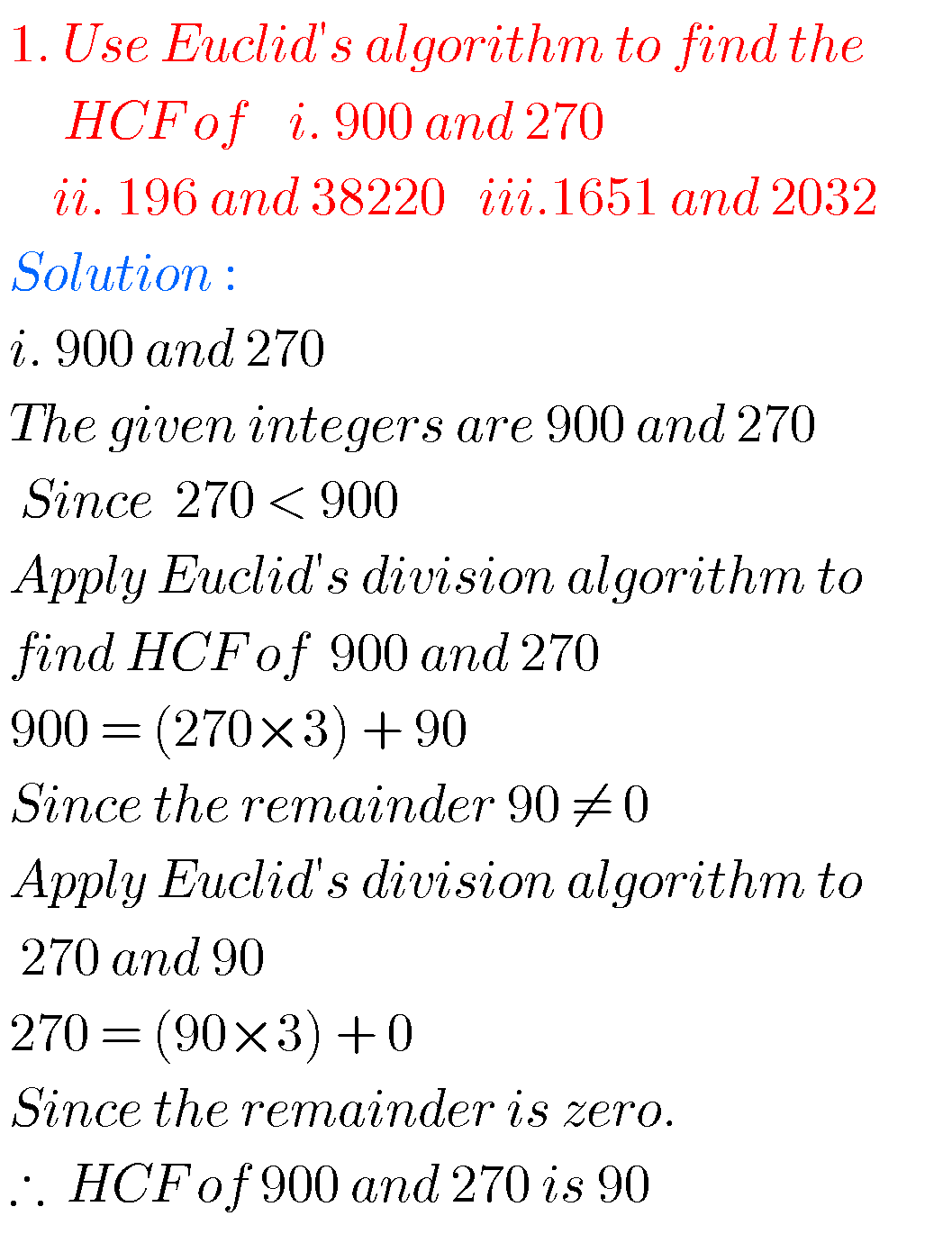##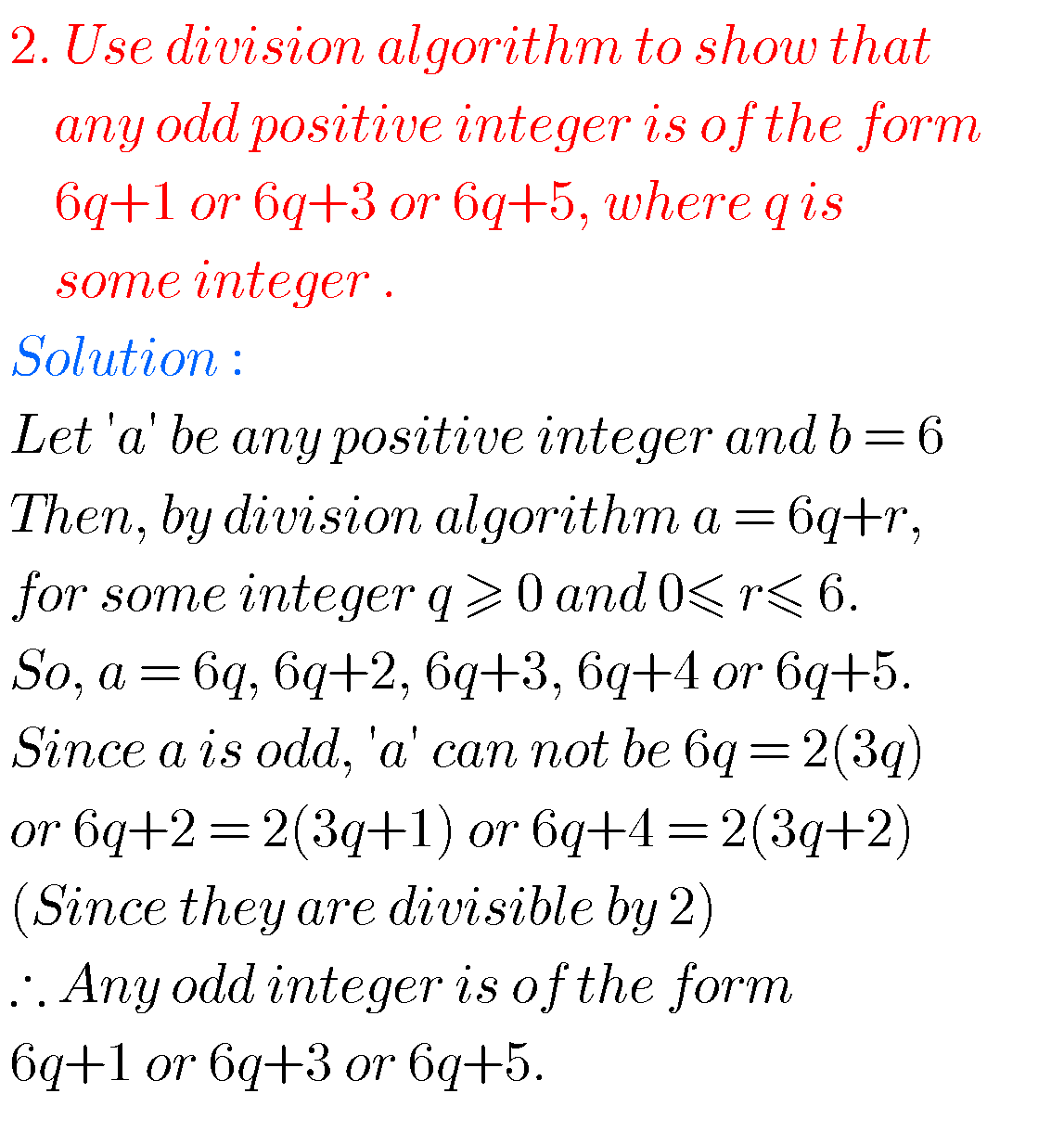Problem 3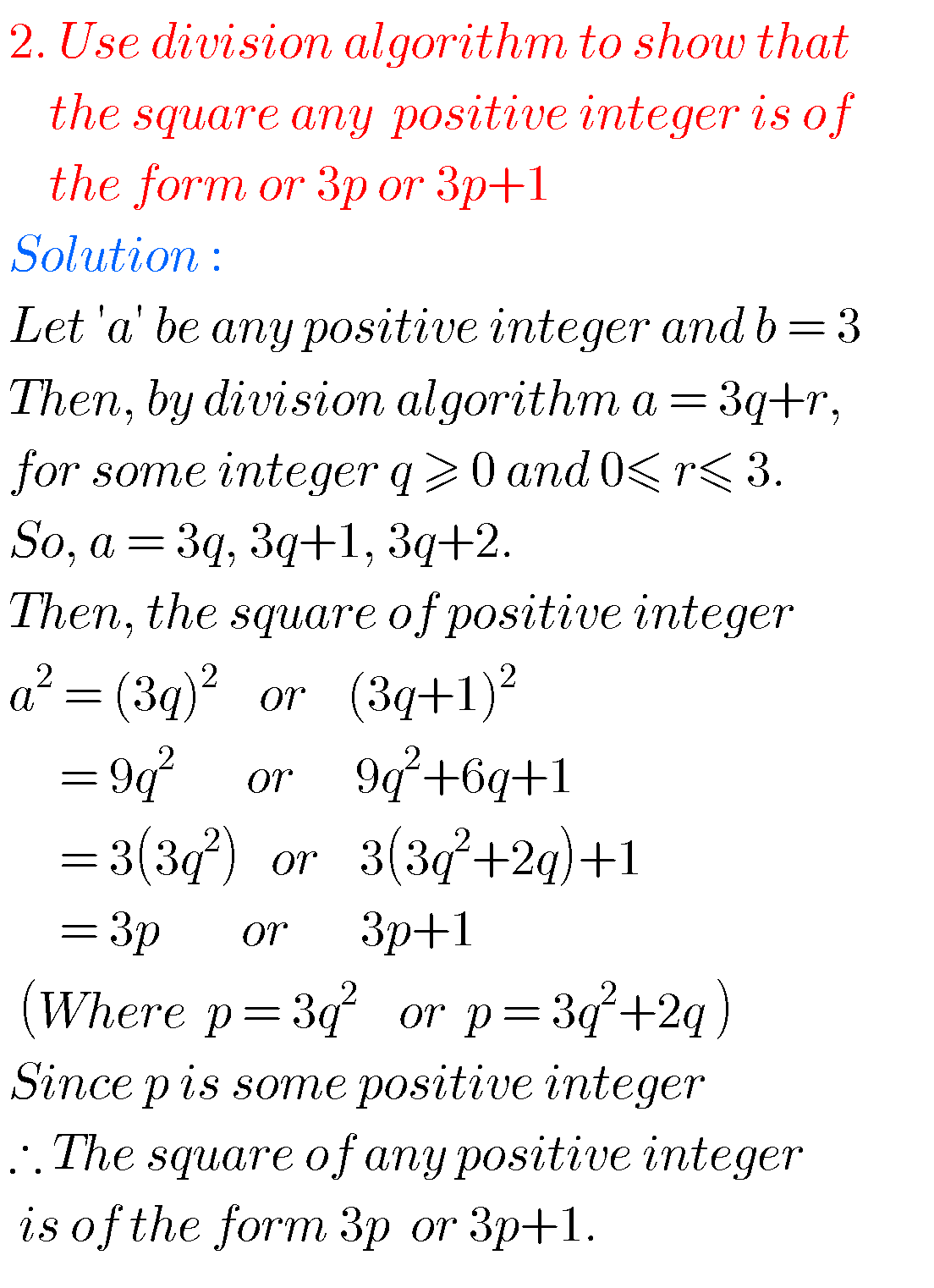## Maths Real numbers – Exercise 1.2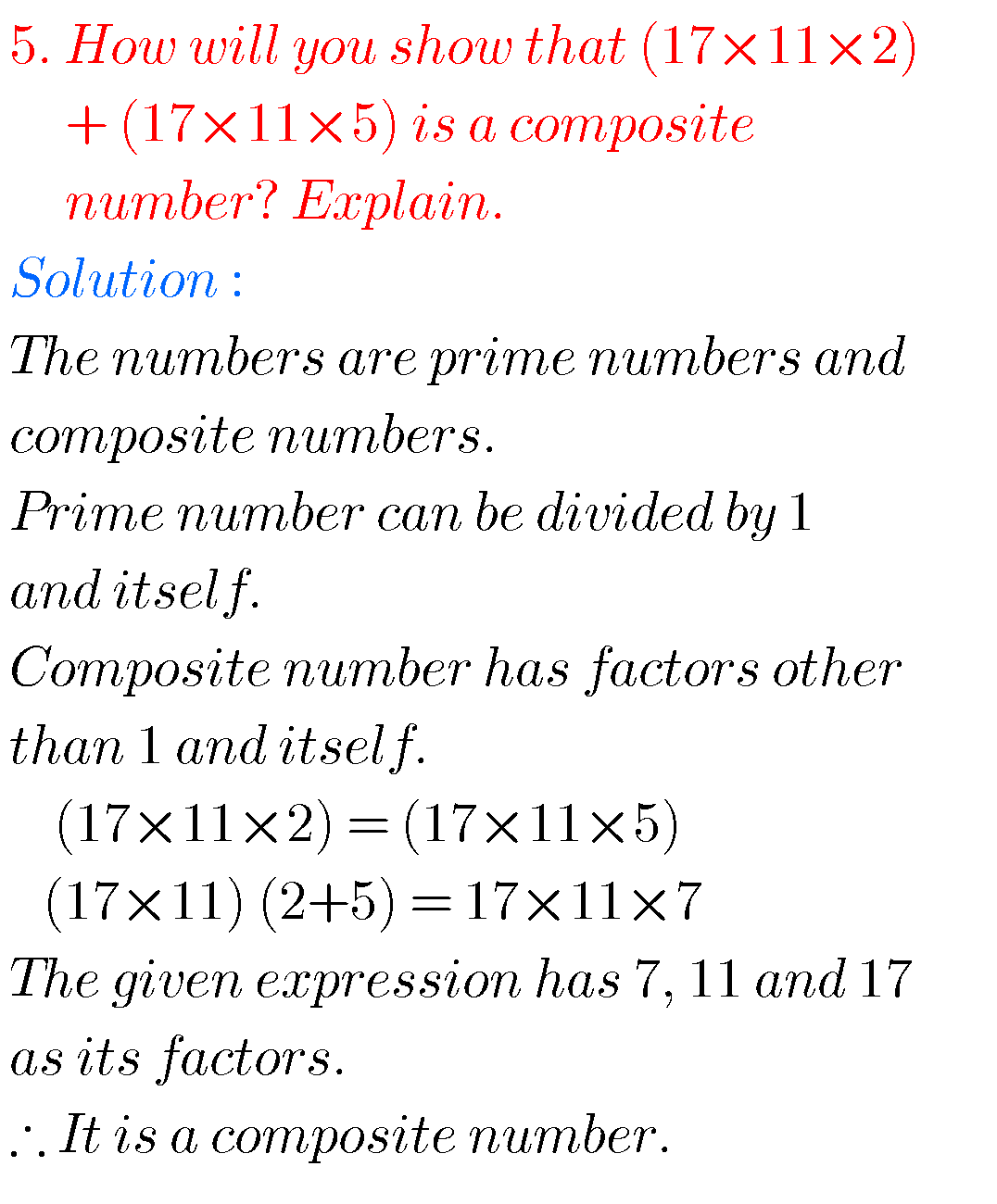### Chapter 1 Real numbers – Exercise 1.3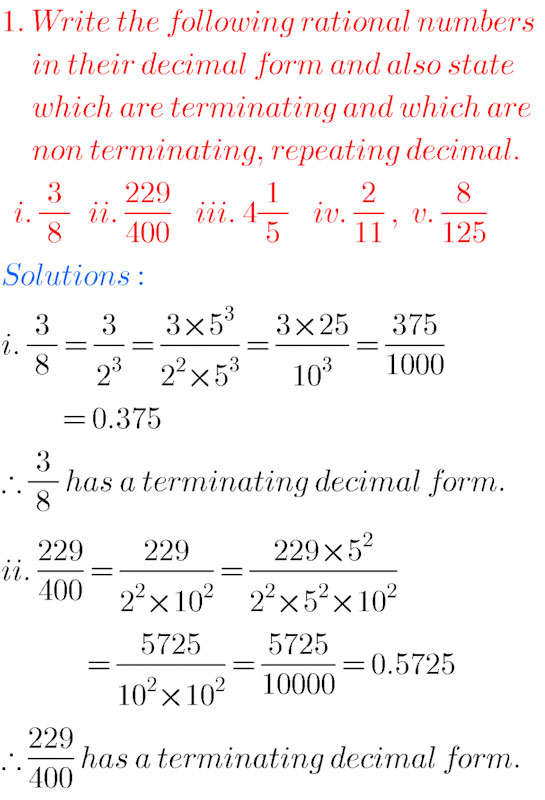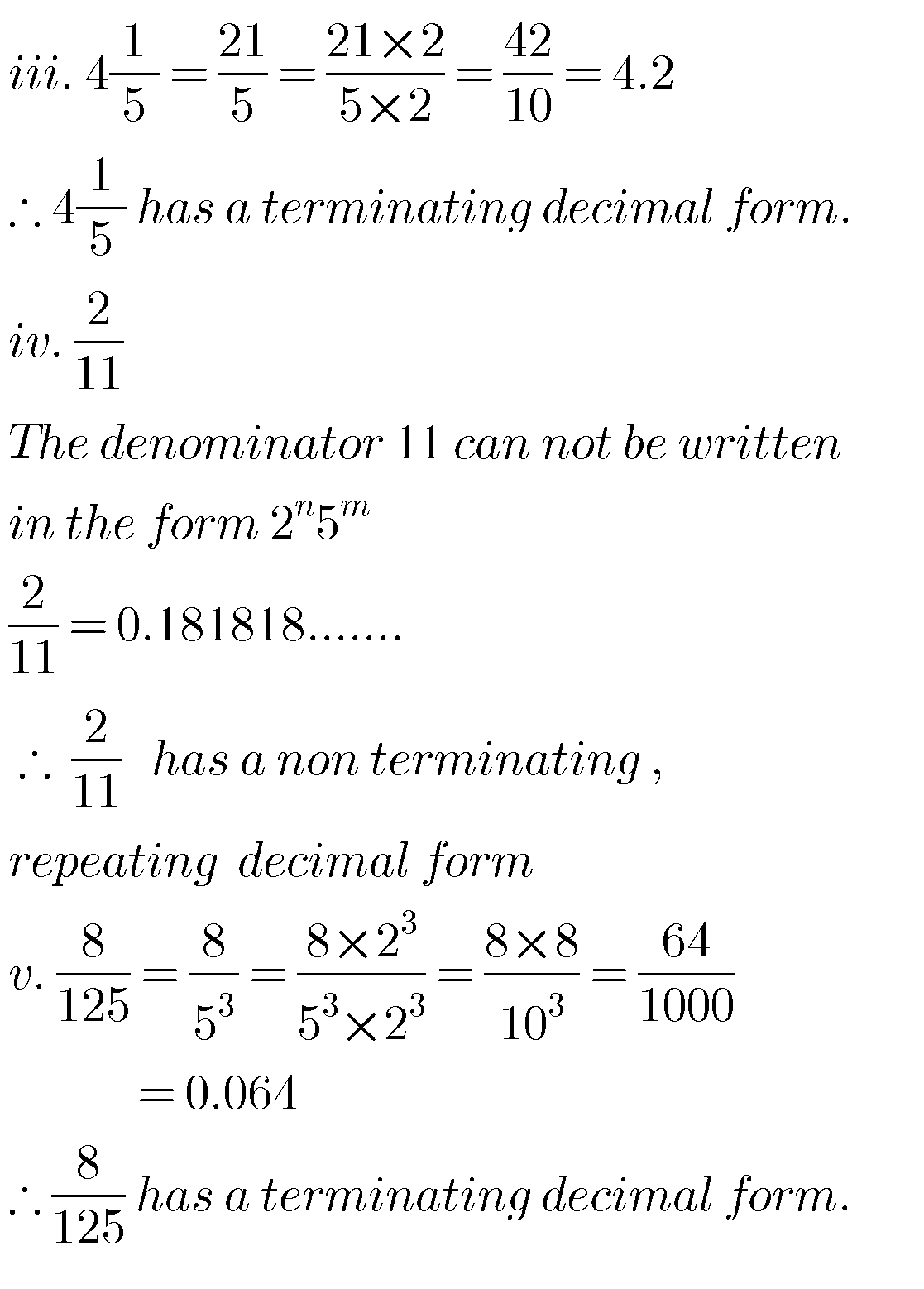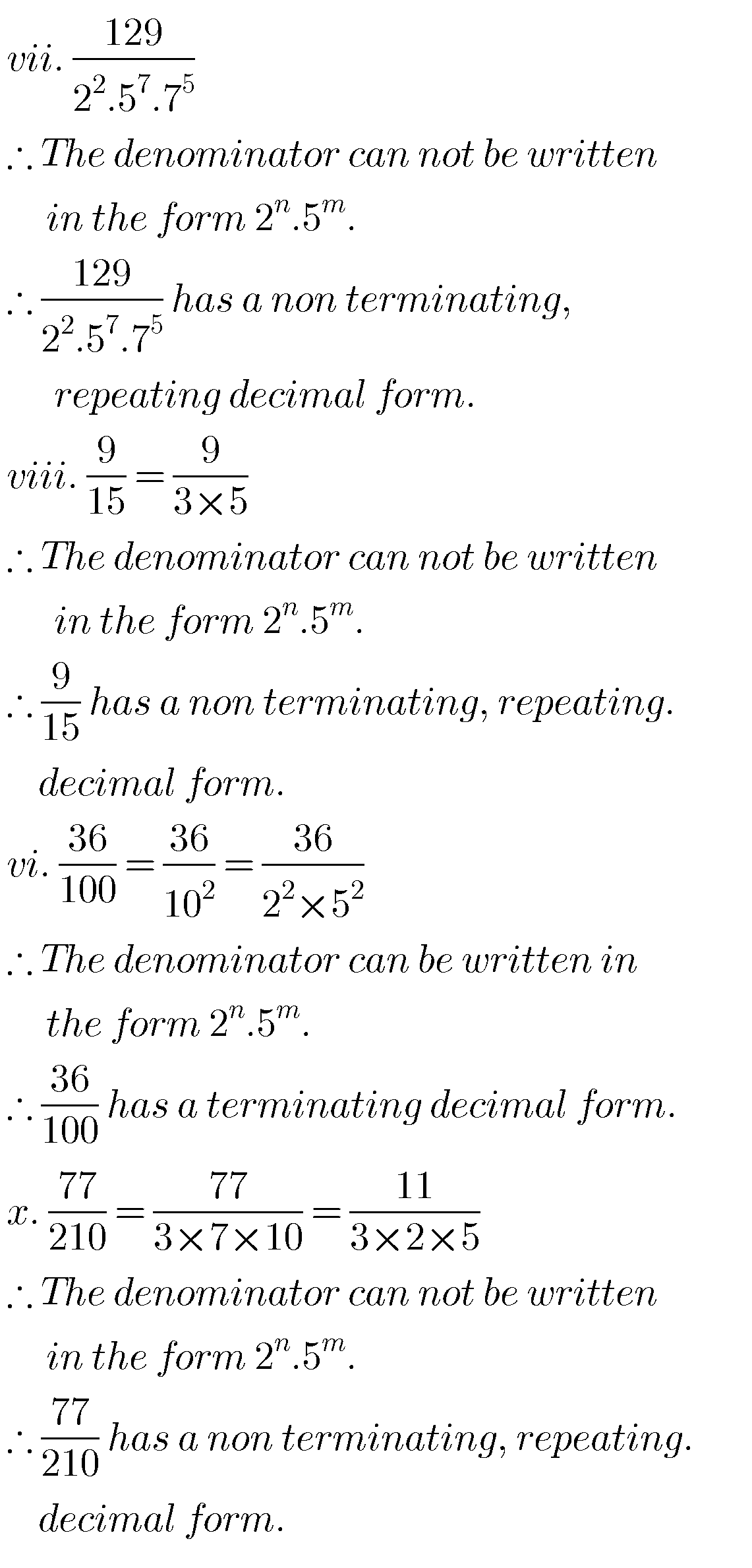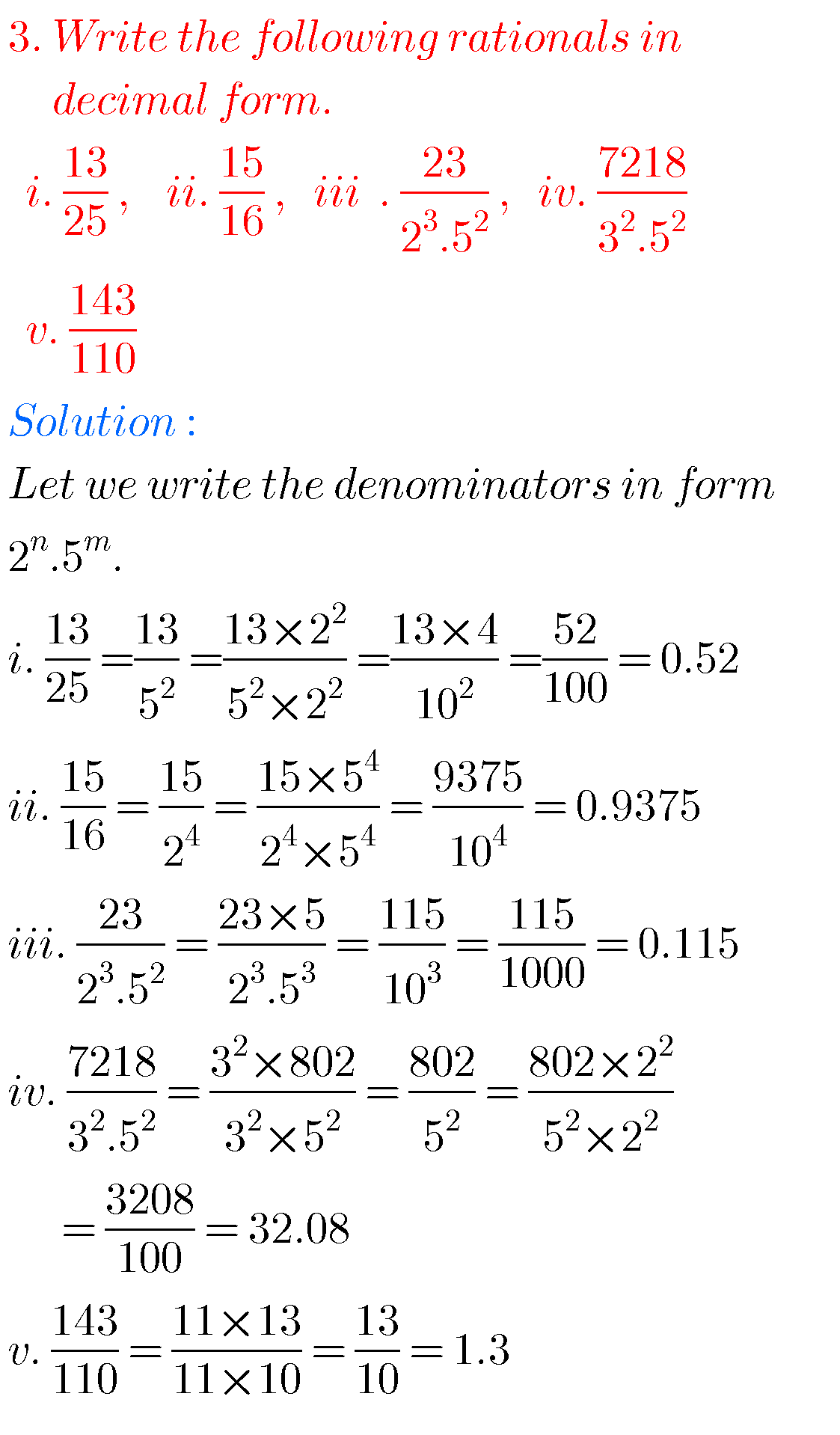###### 10 th class Real numbers – Exercise 1.4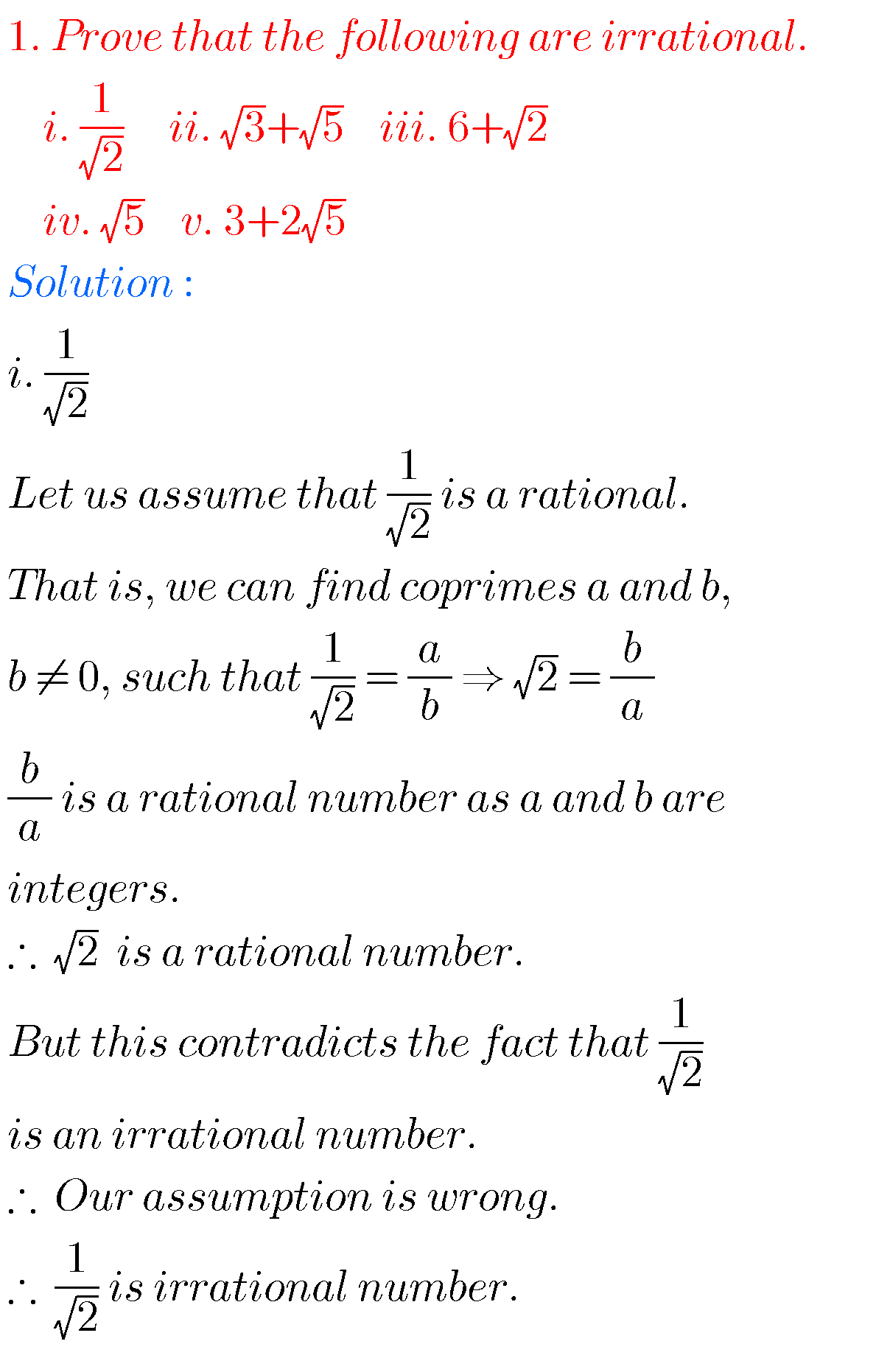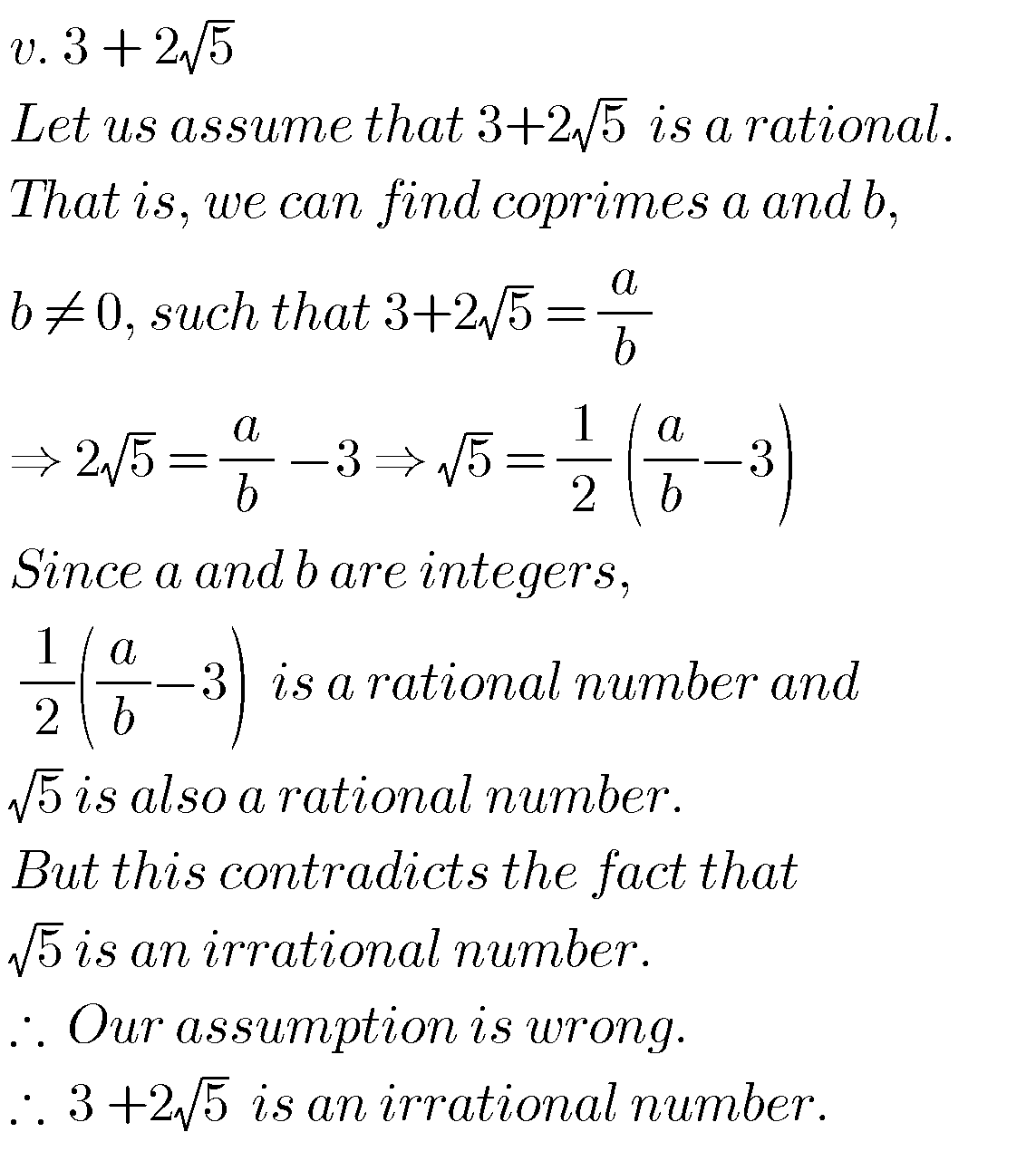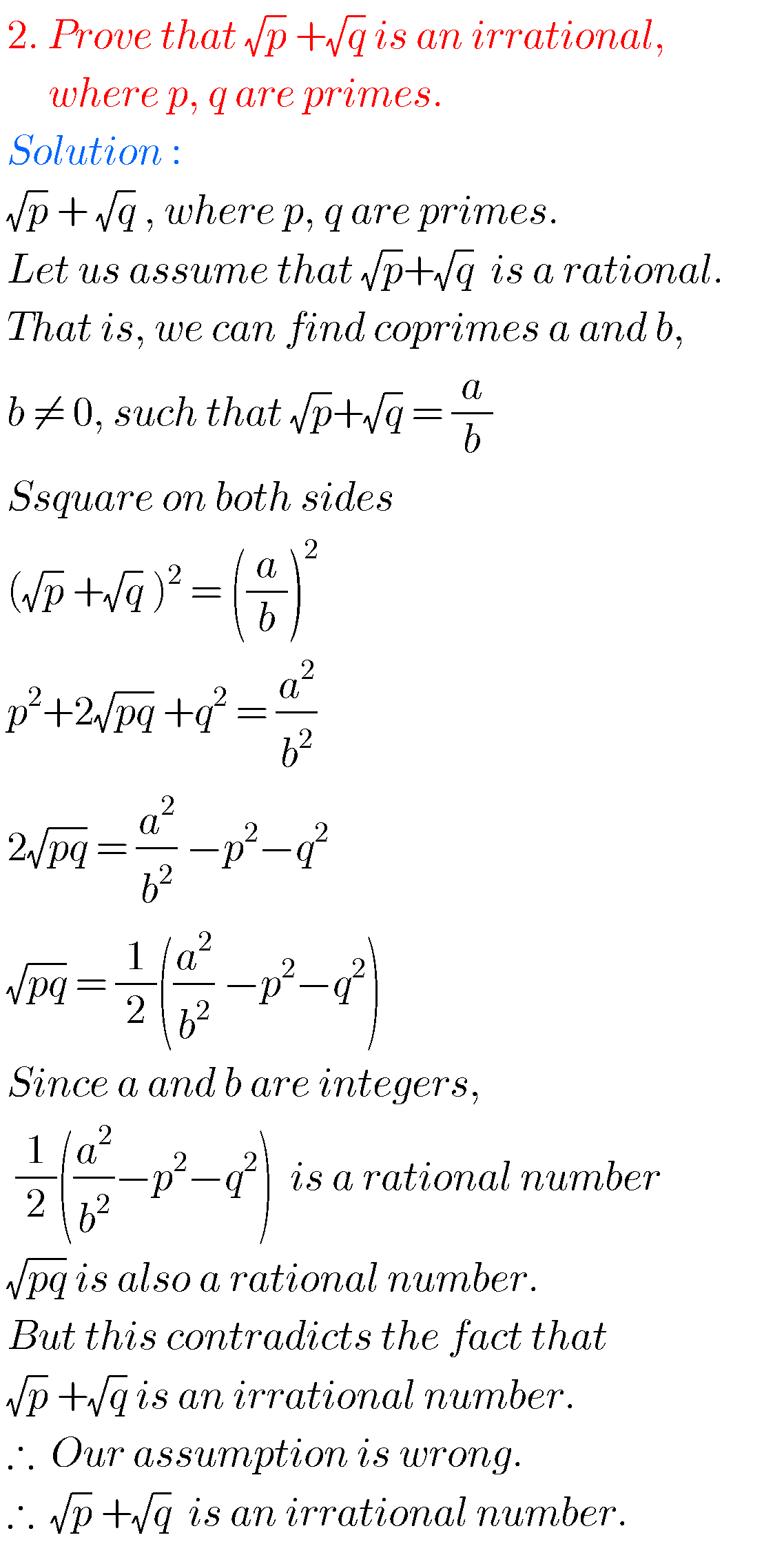## SSC Real numbers – Exercise 1.5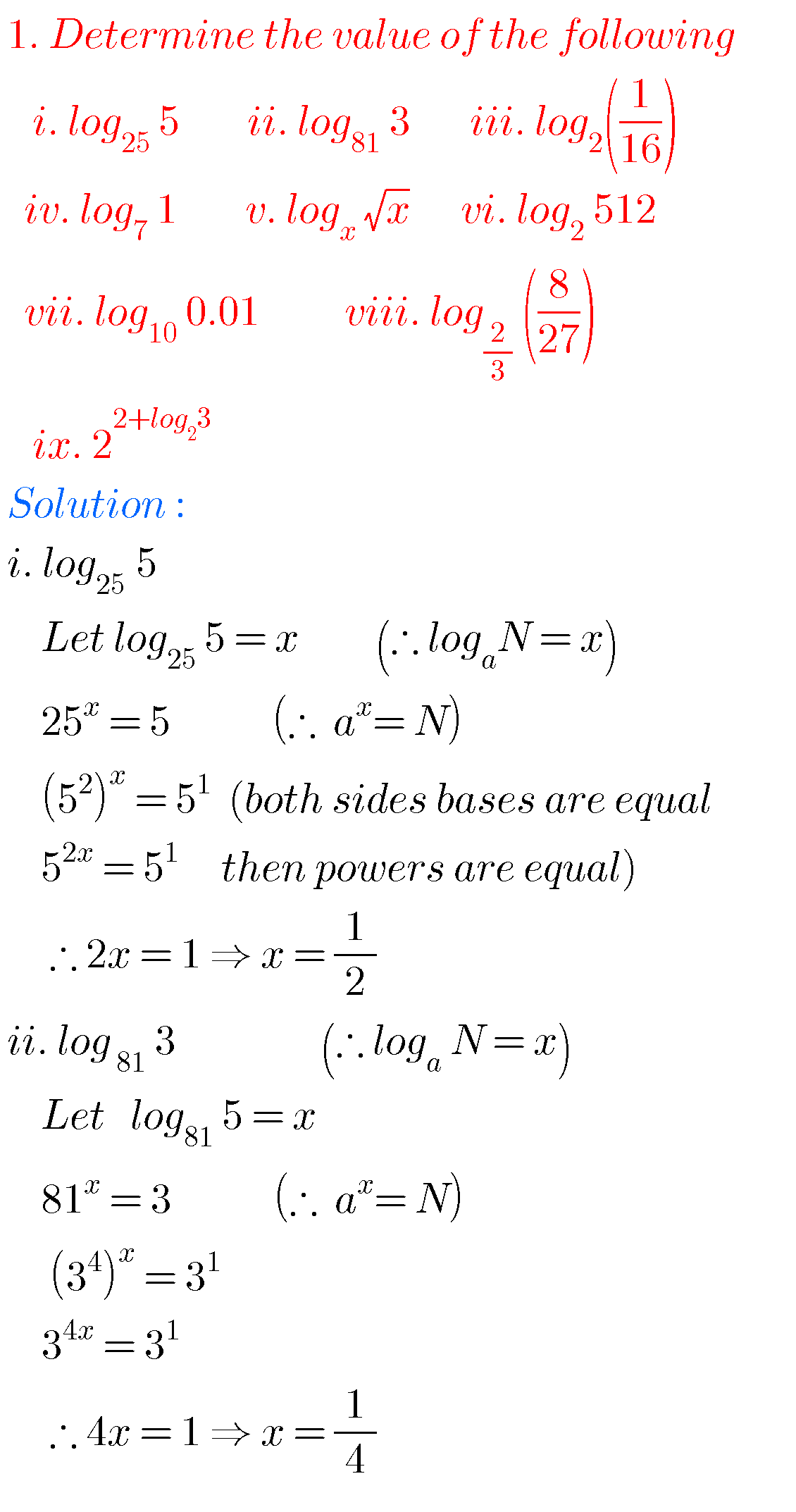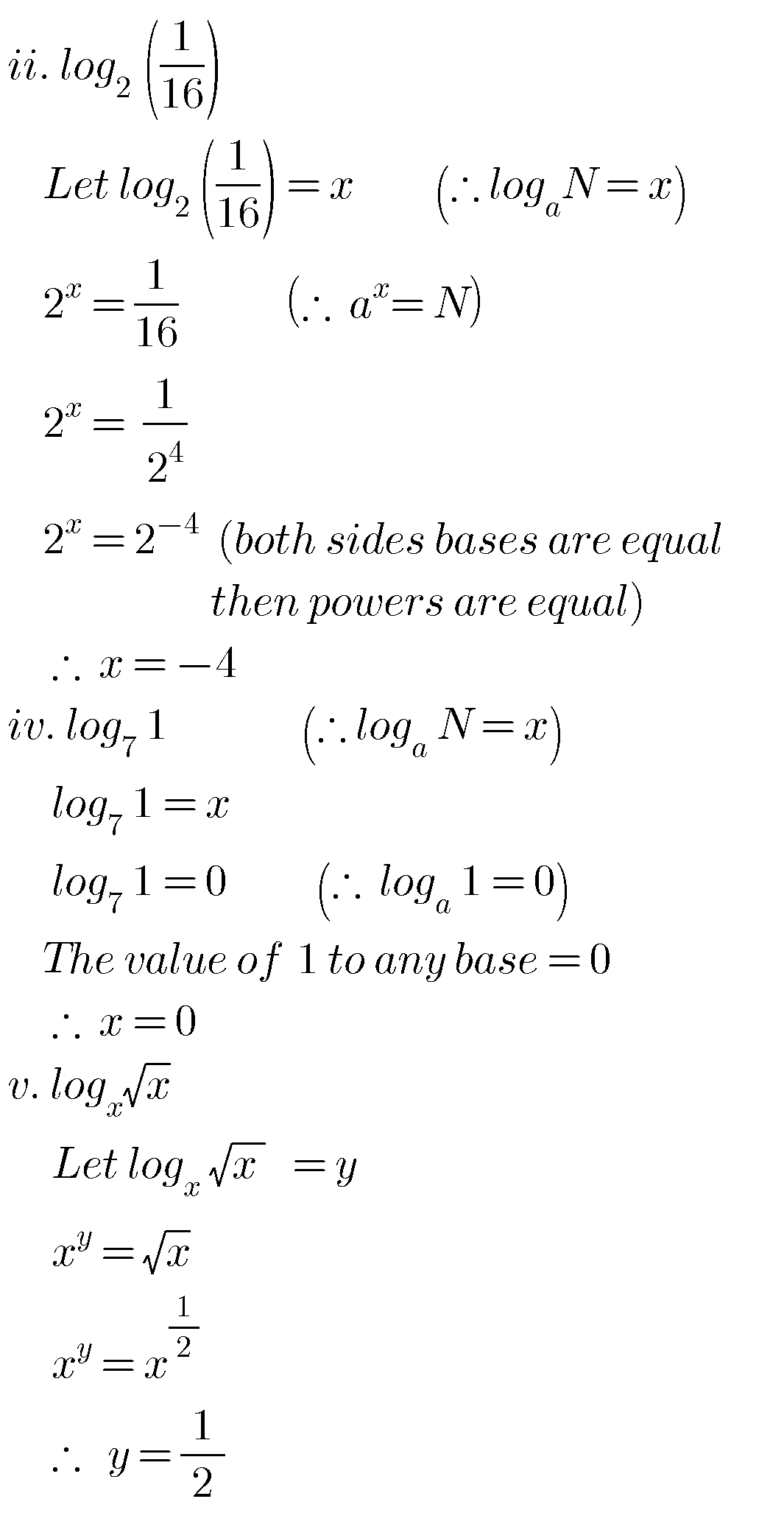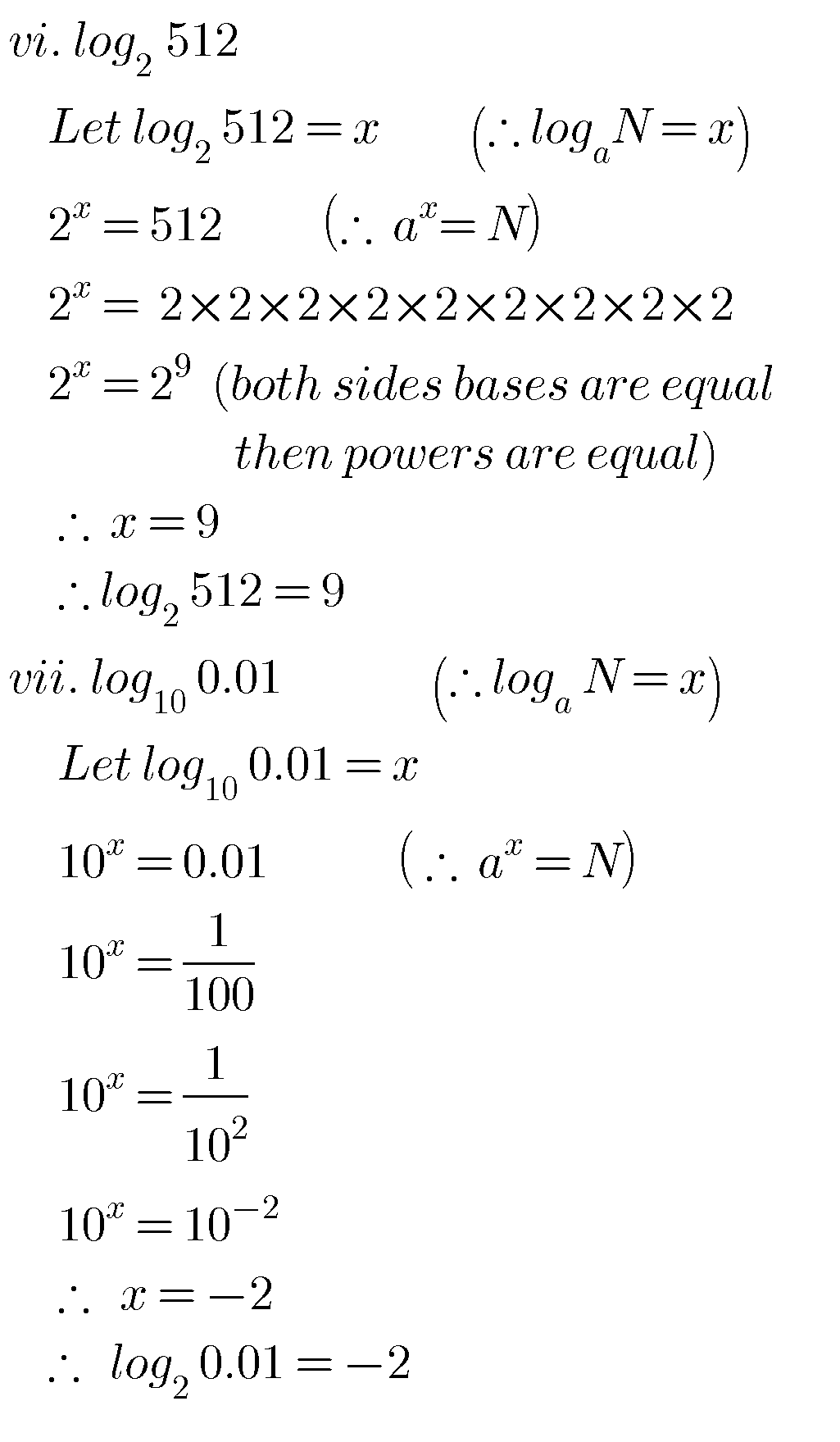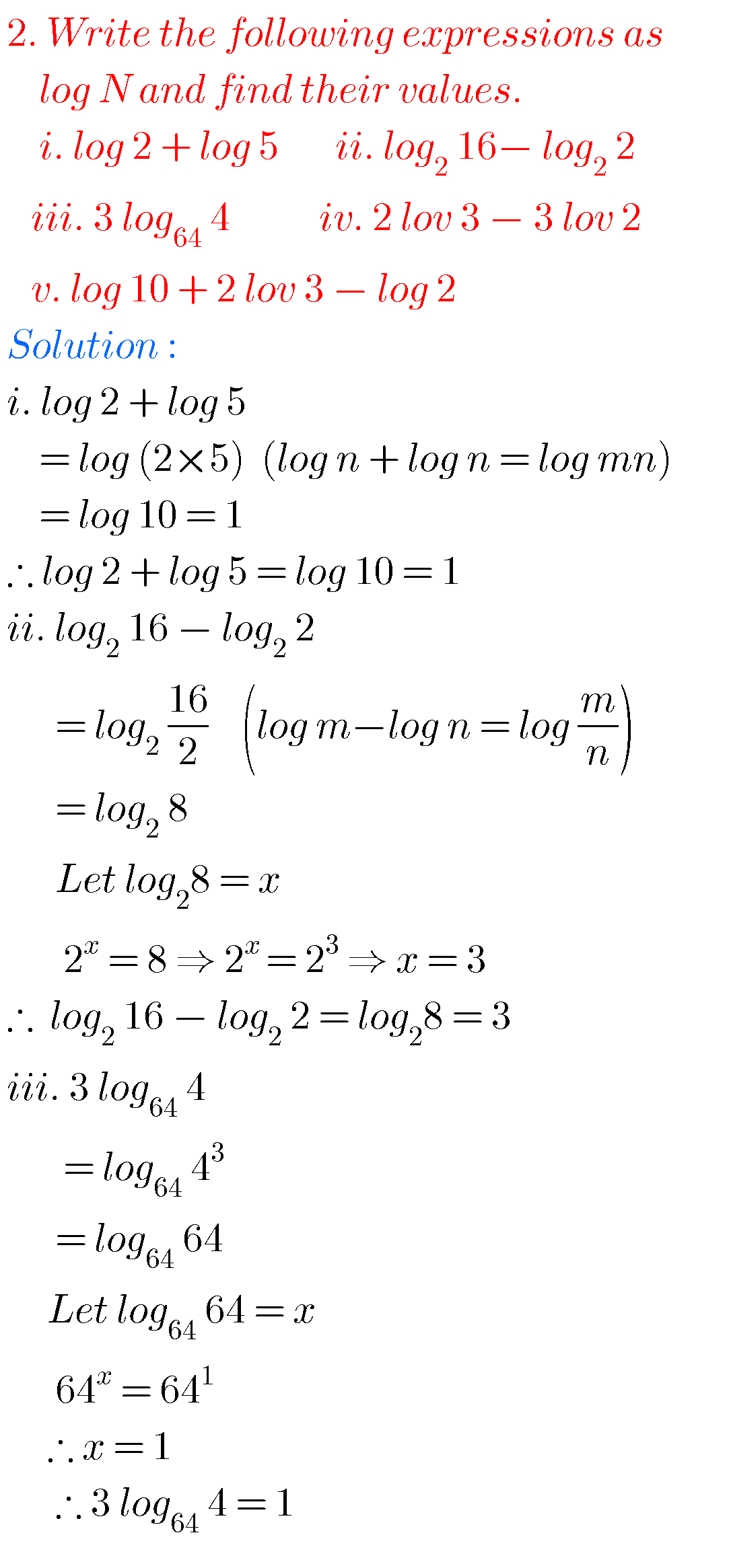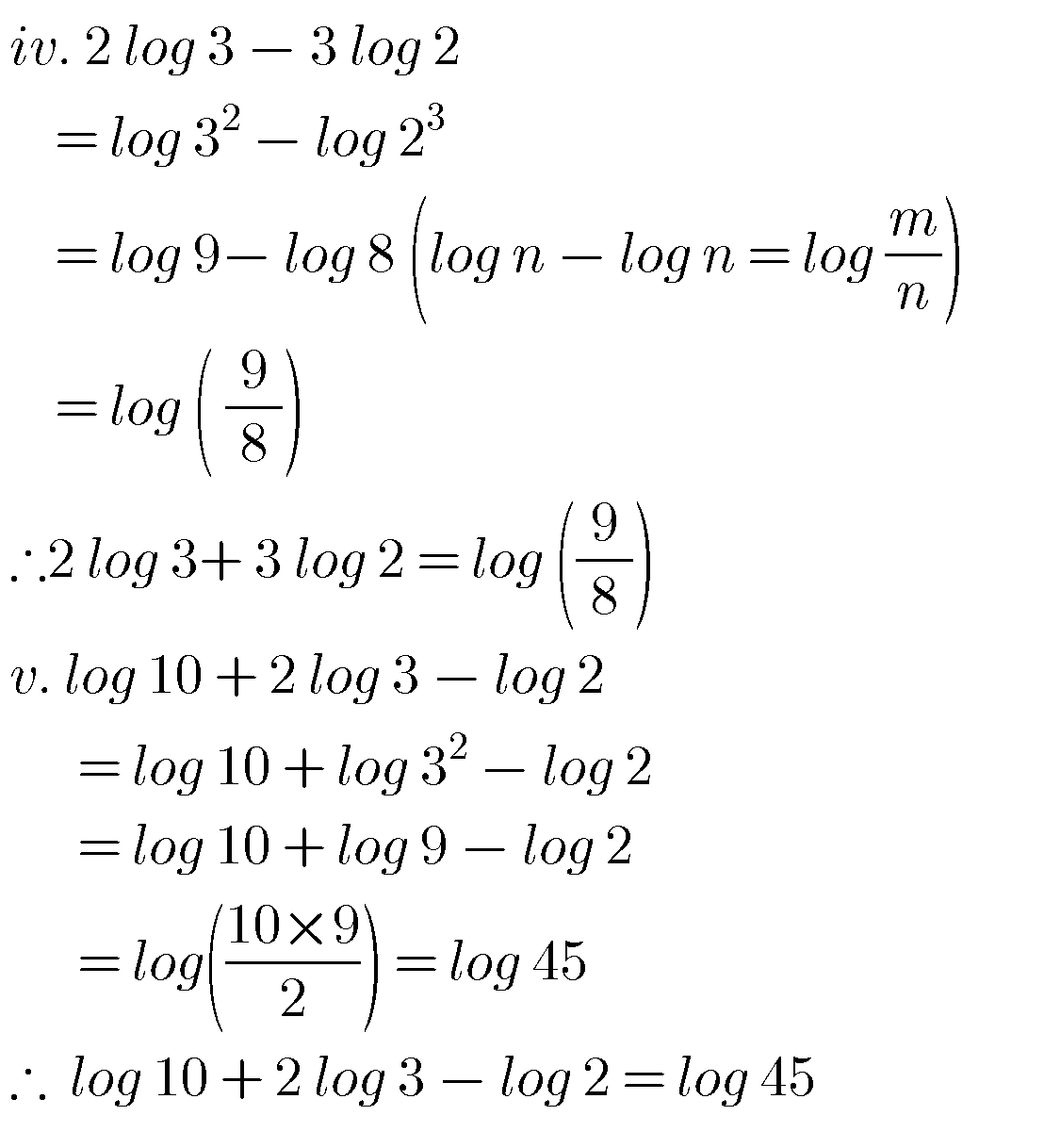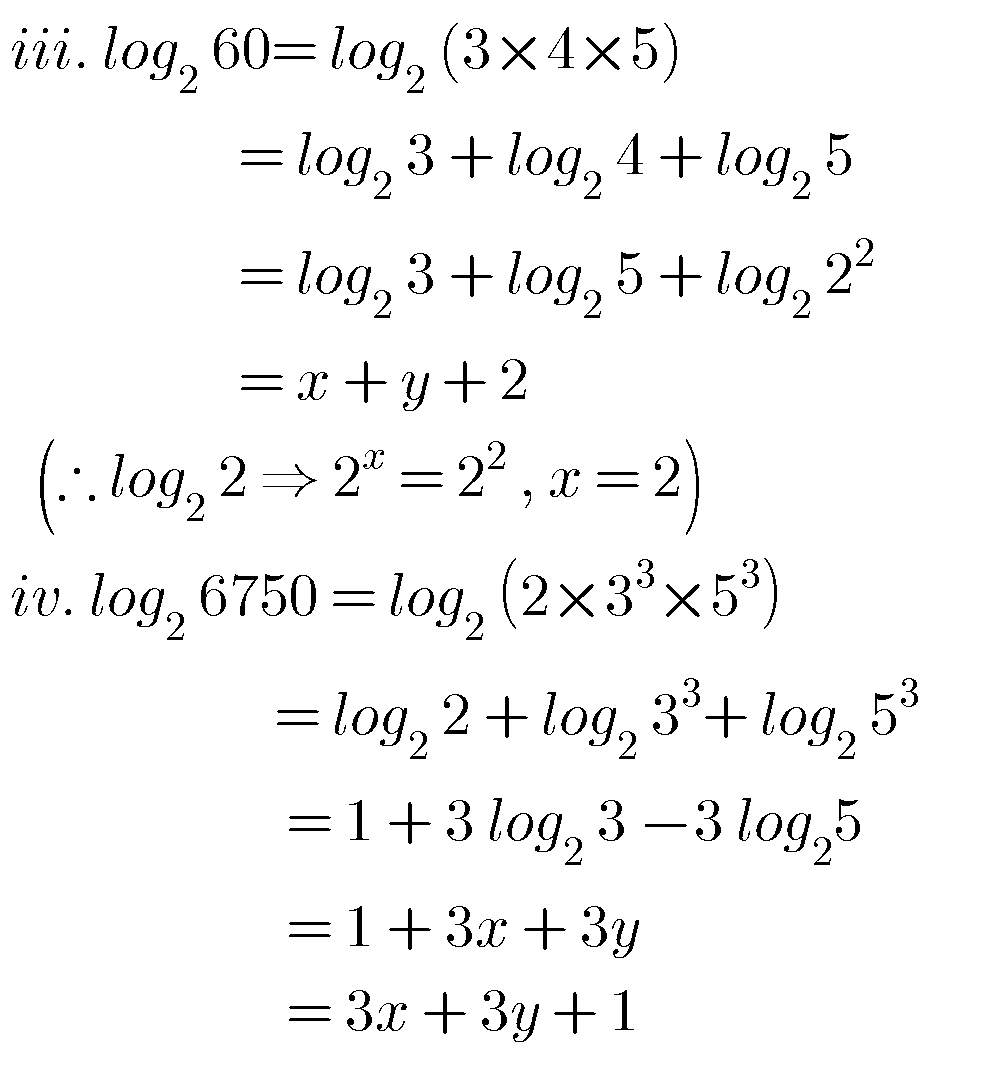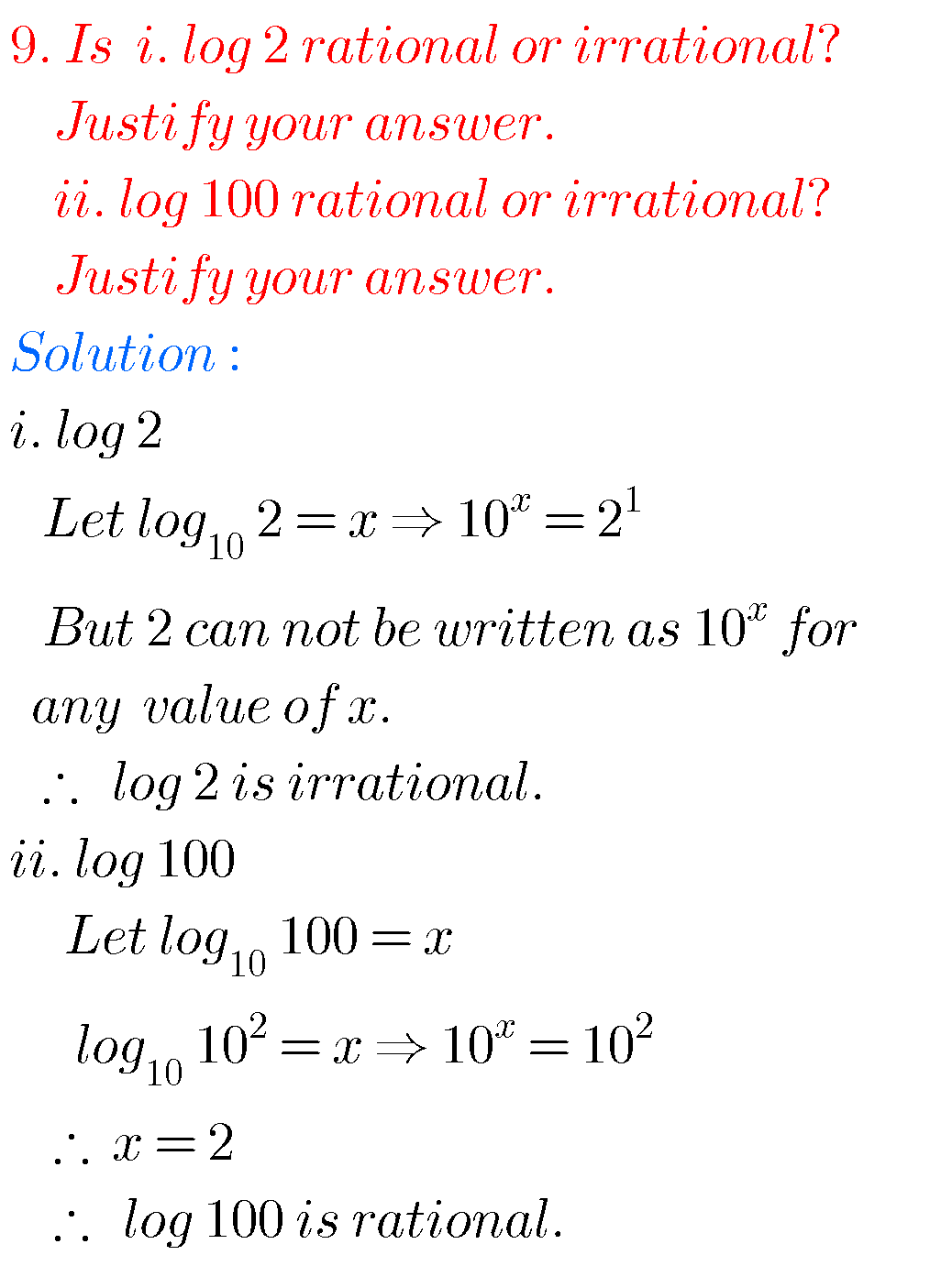Some more solutions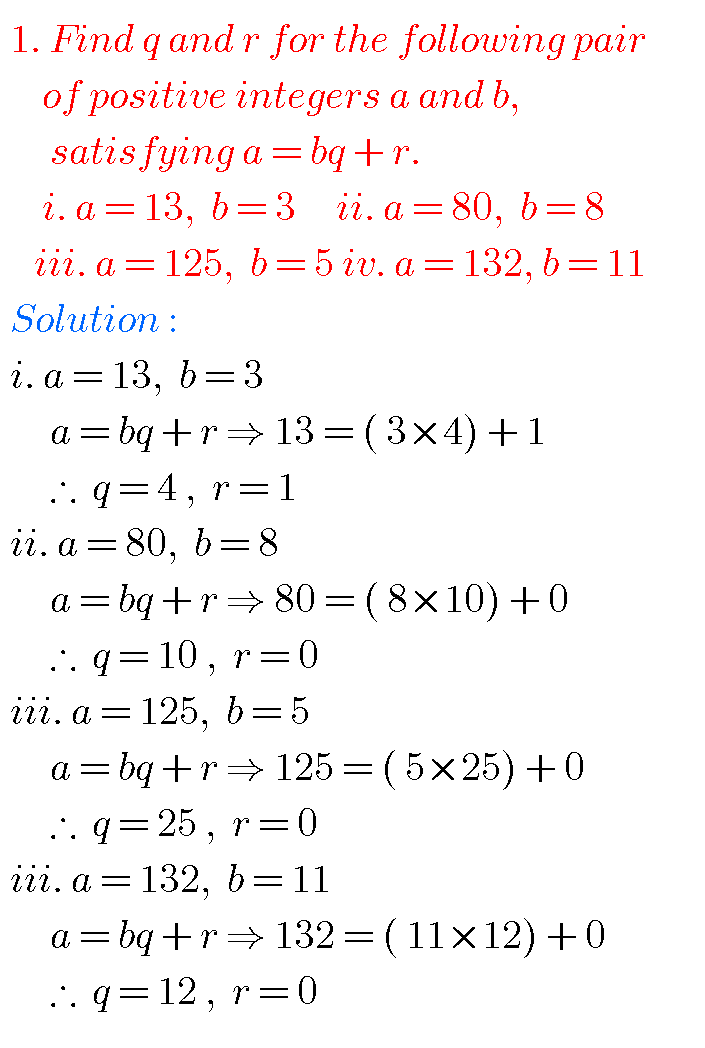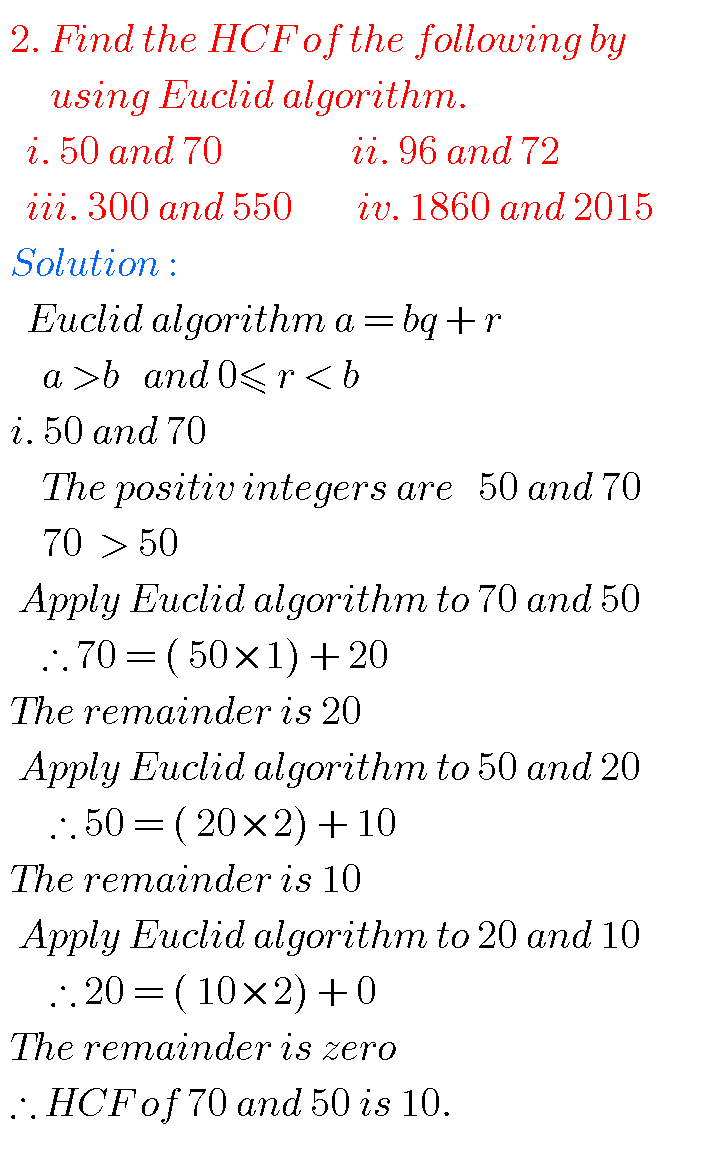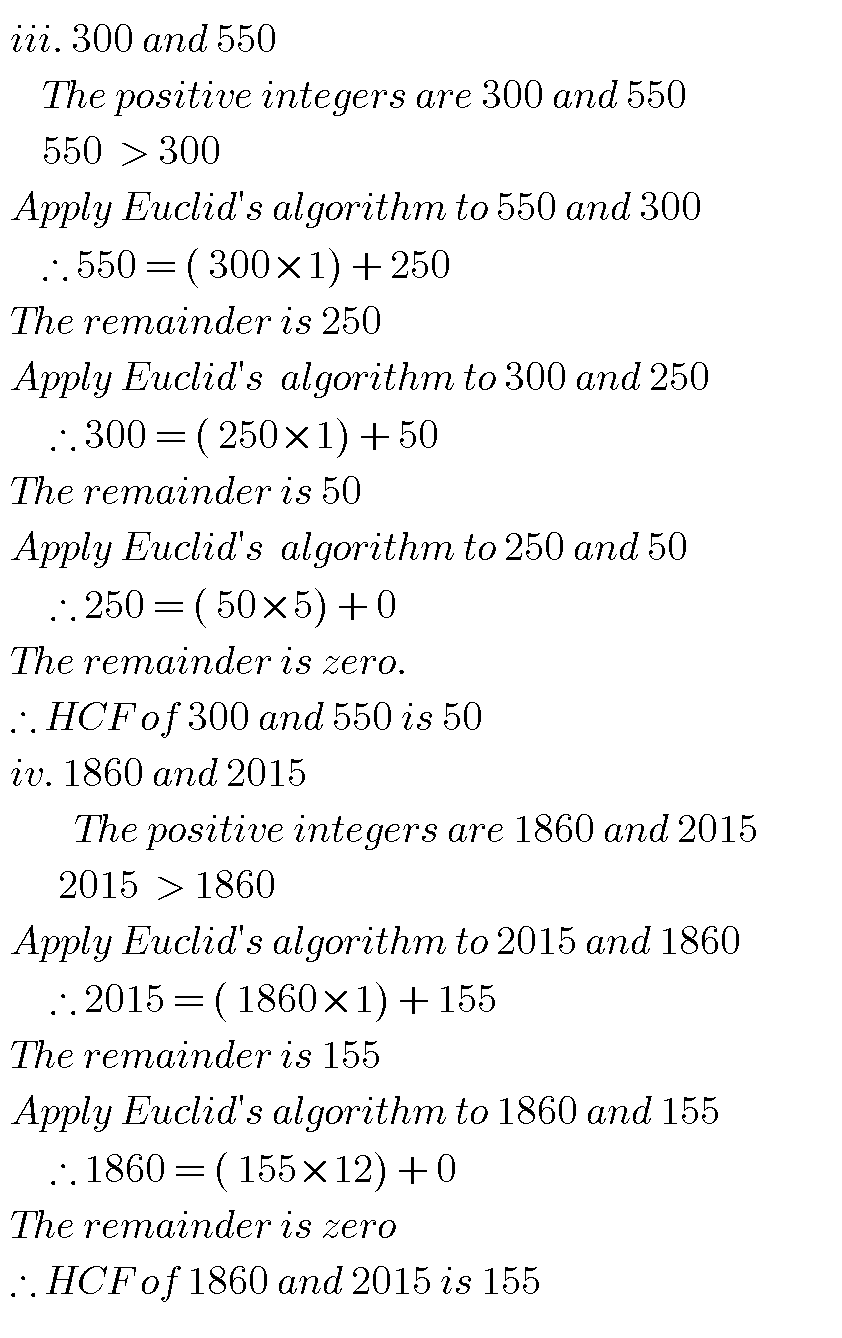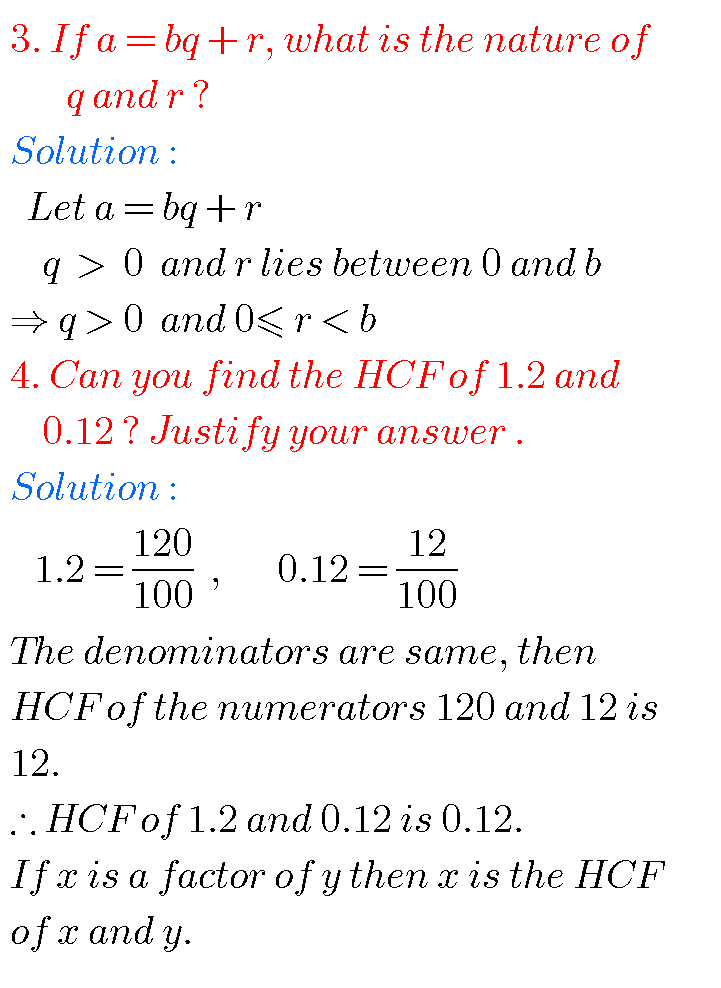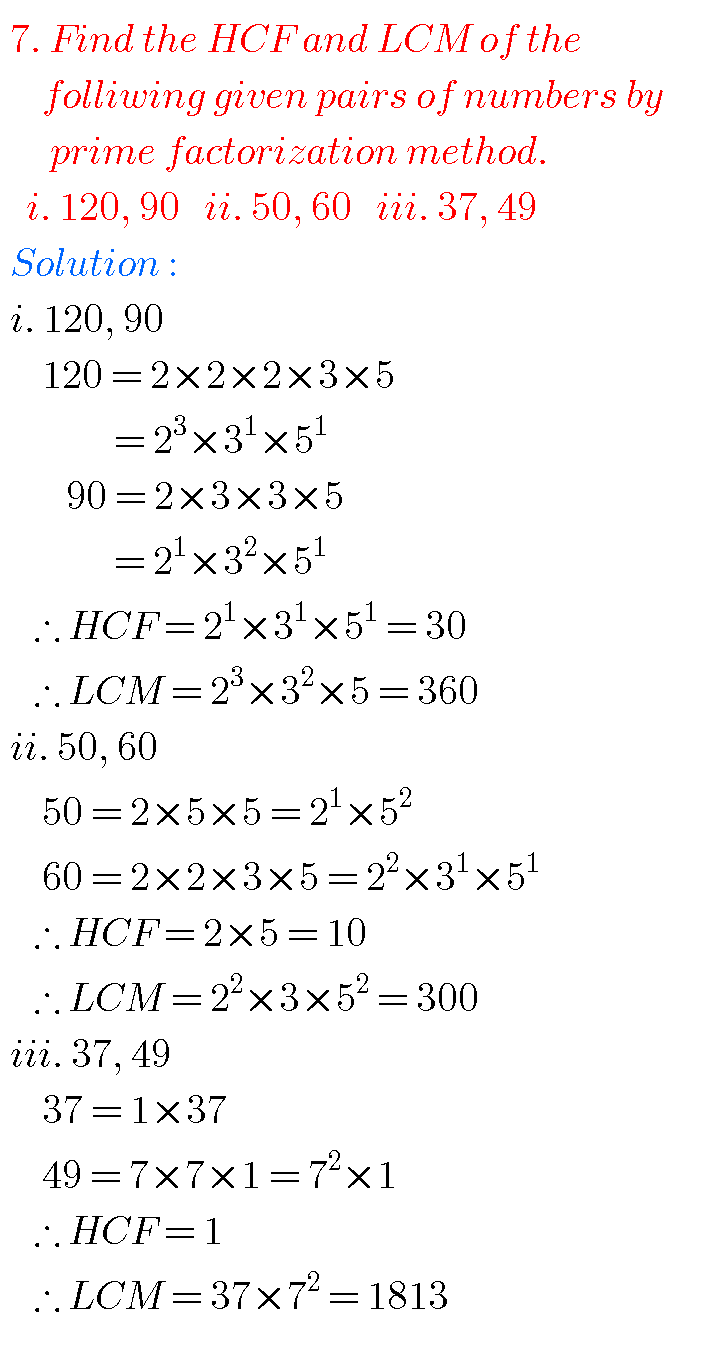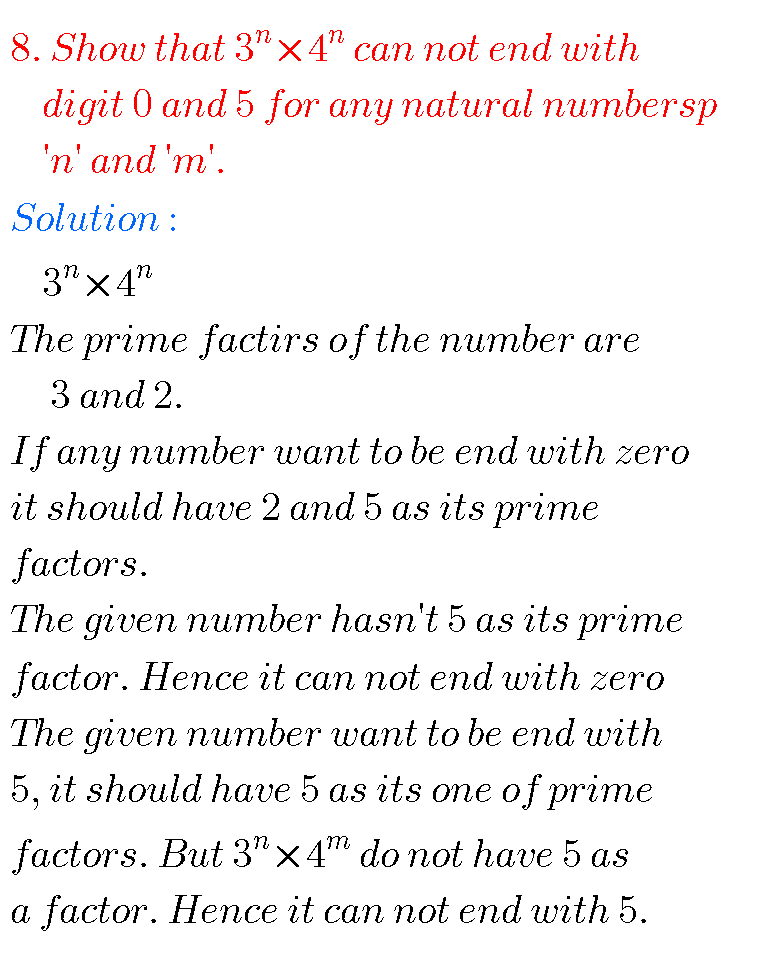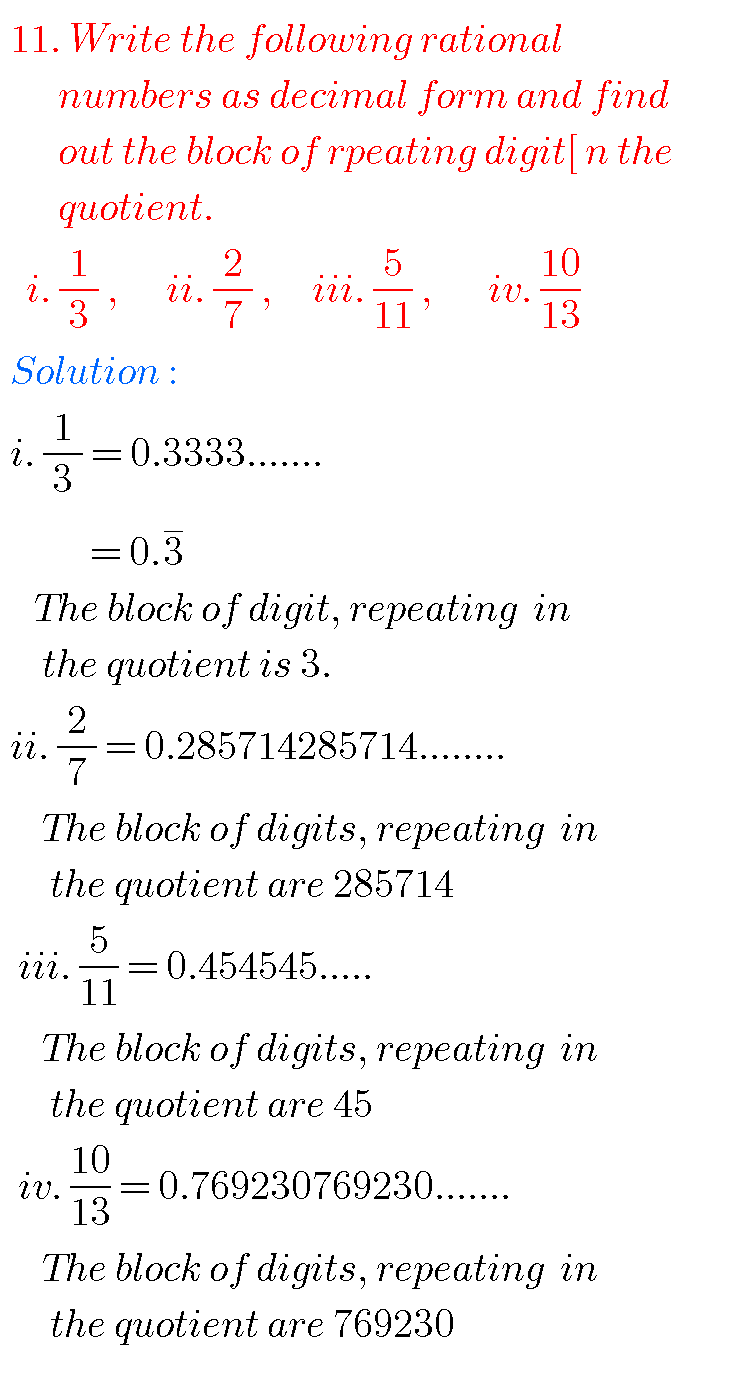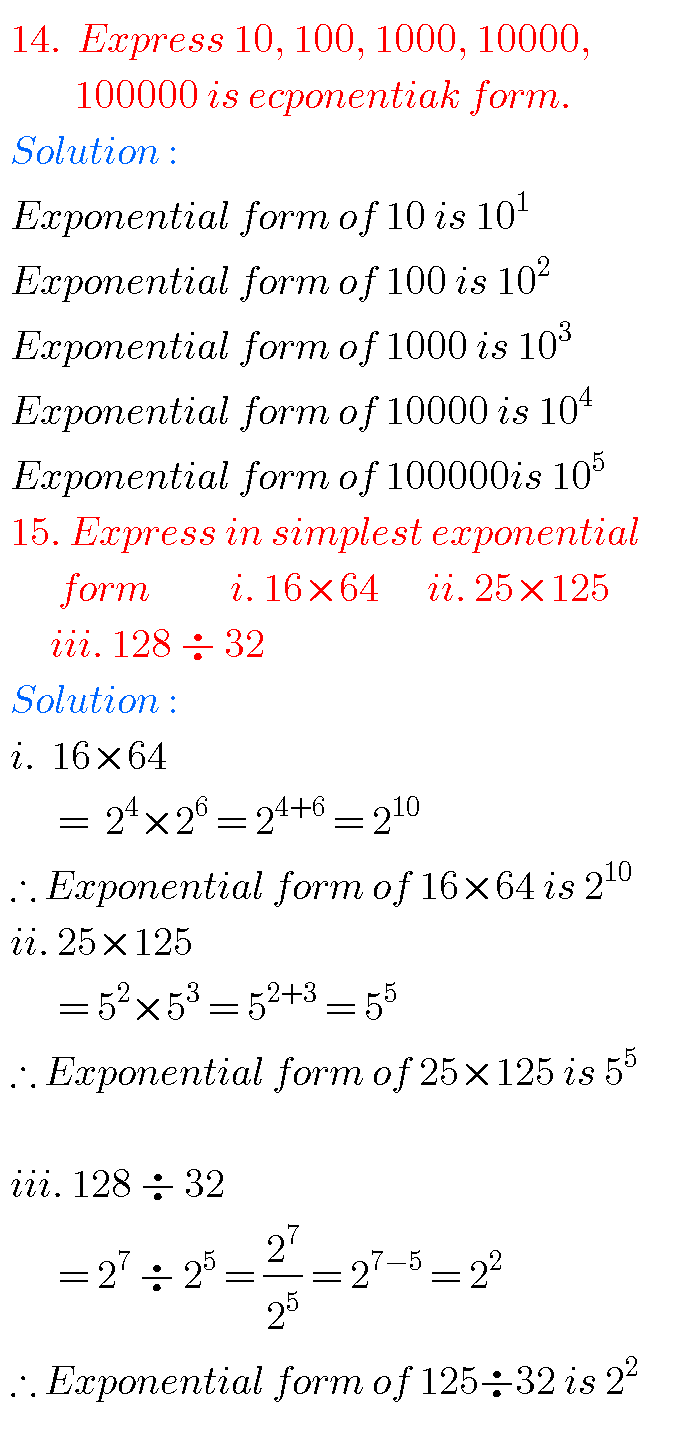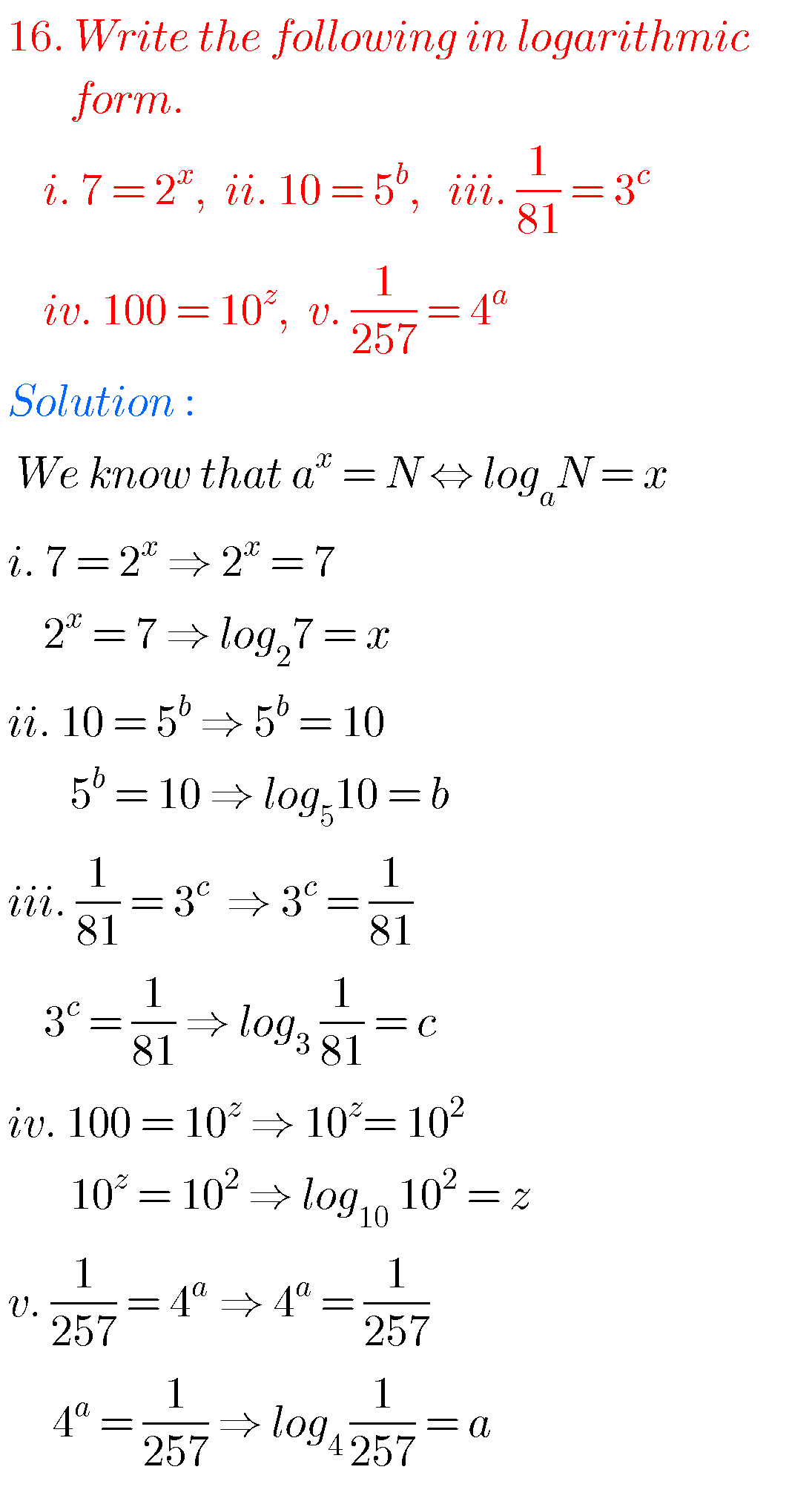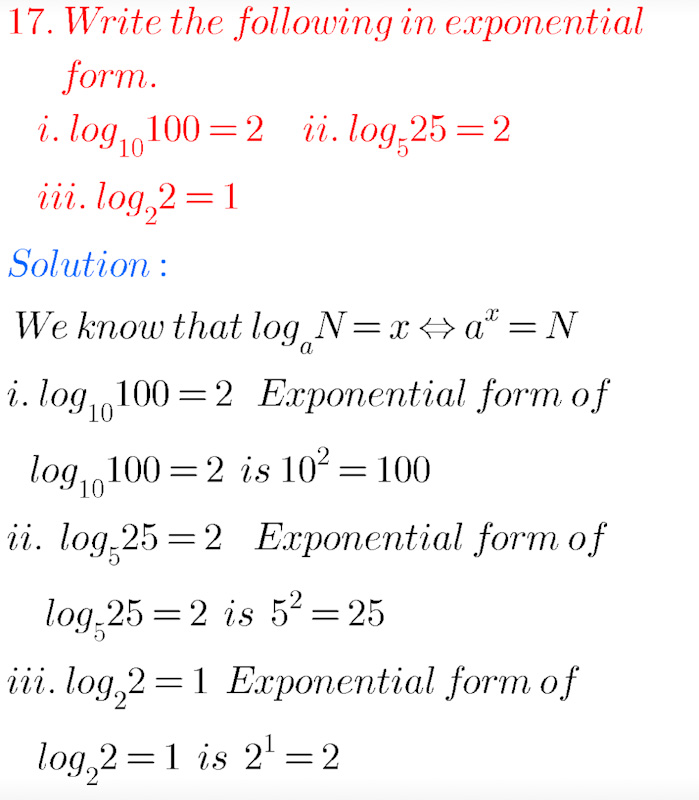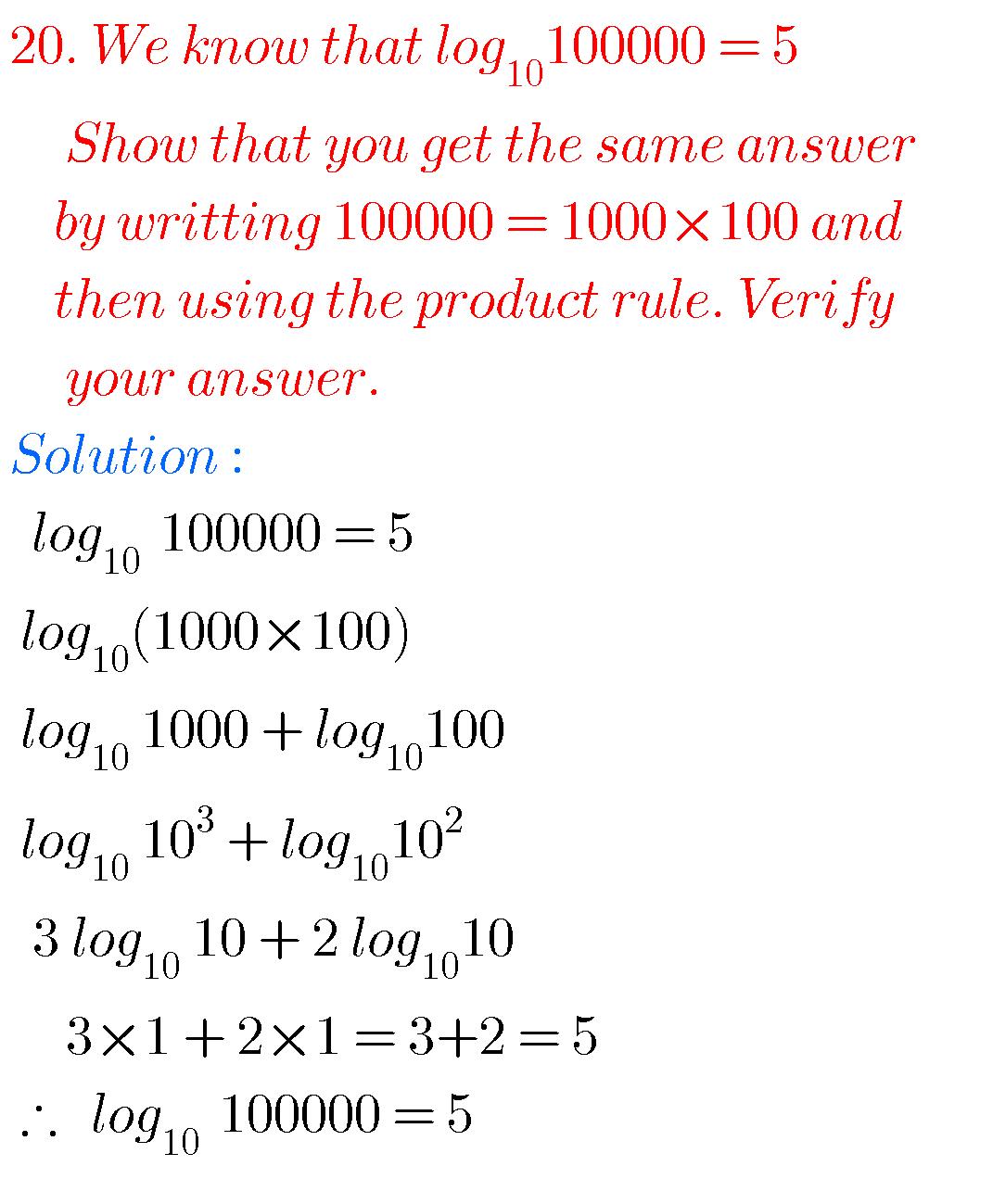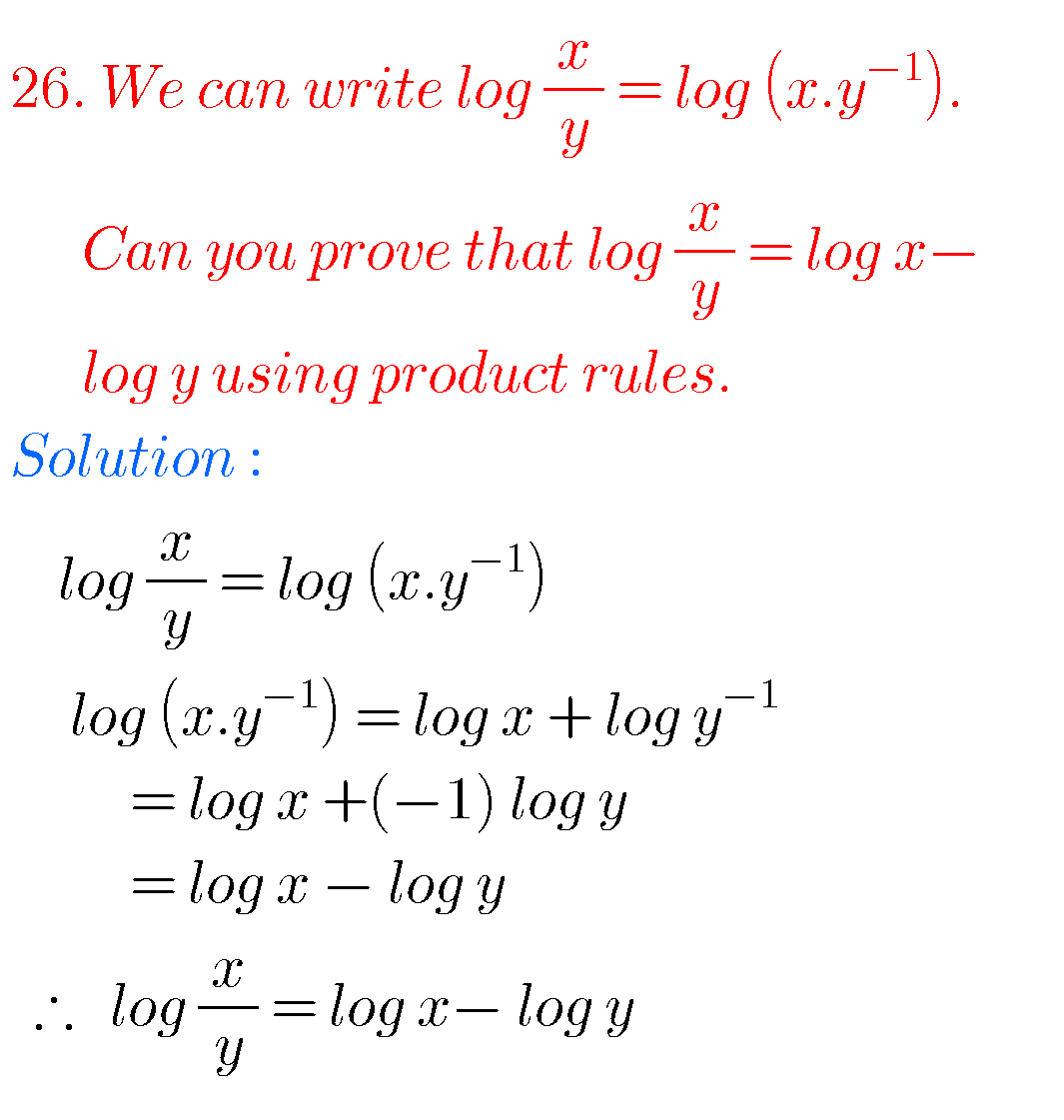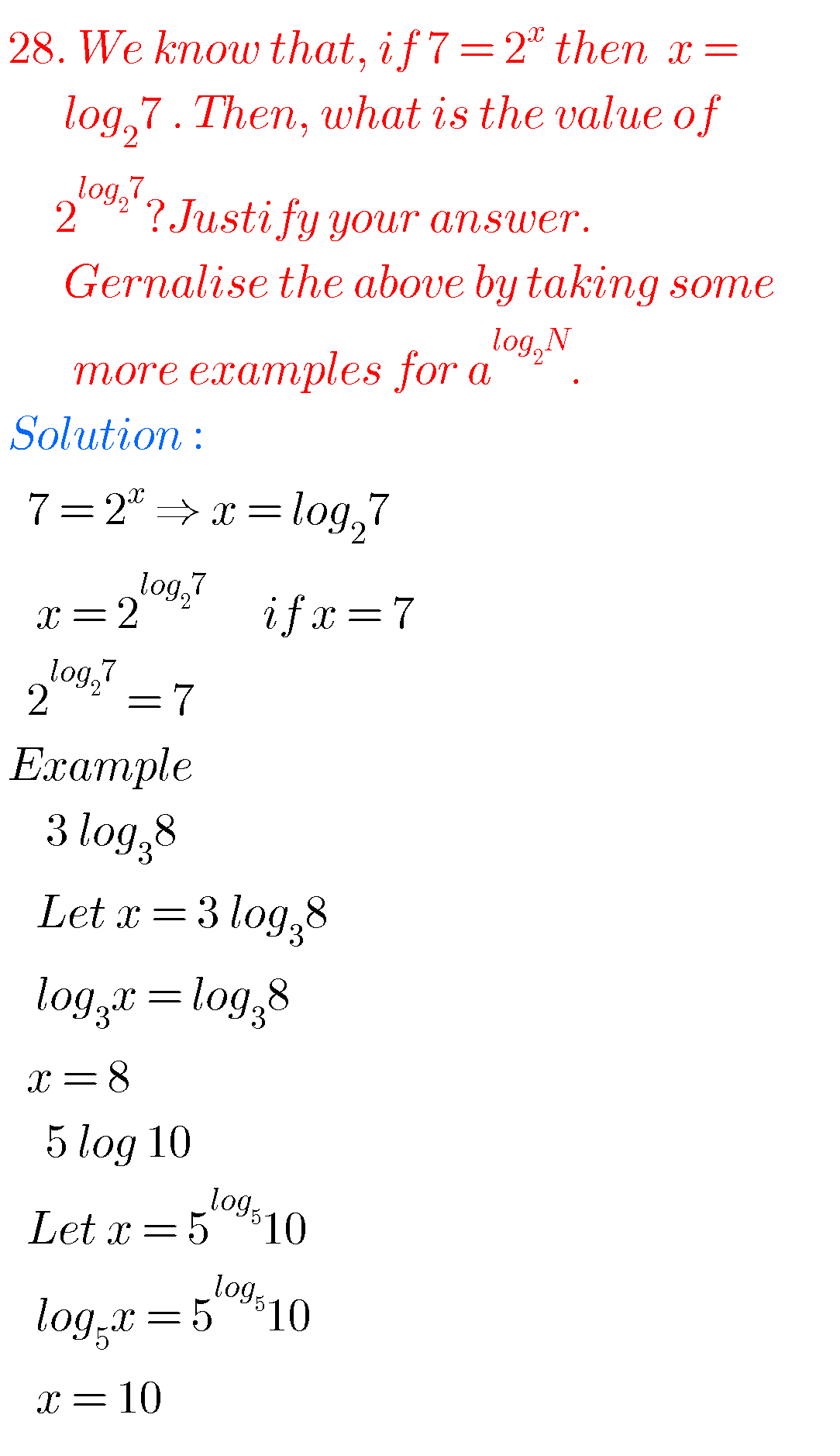Optional exercise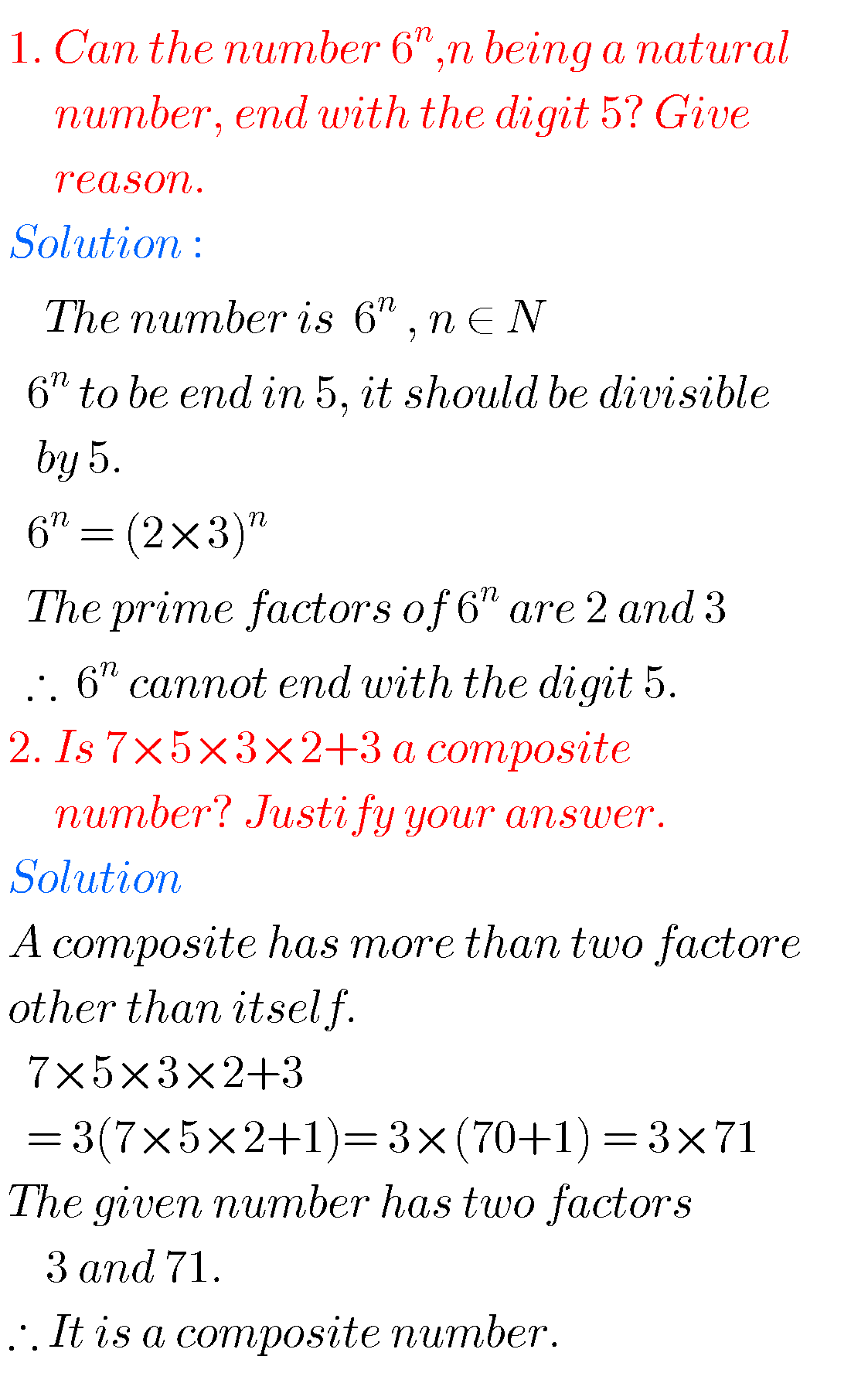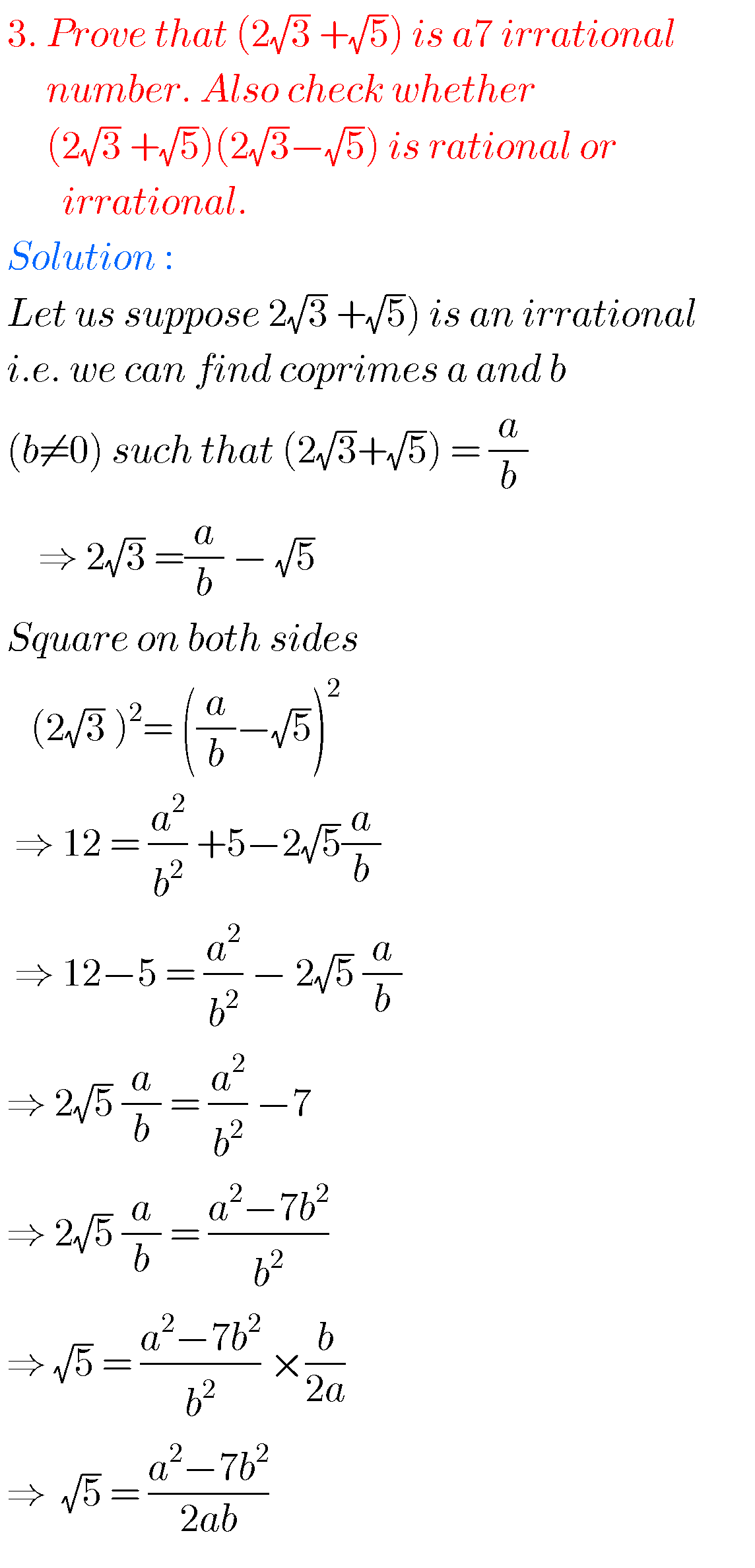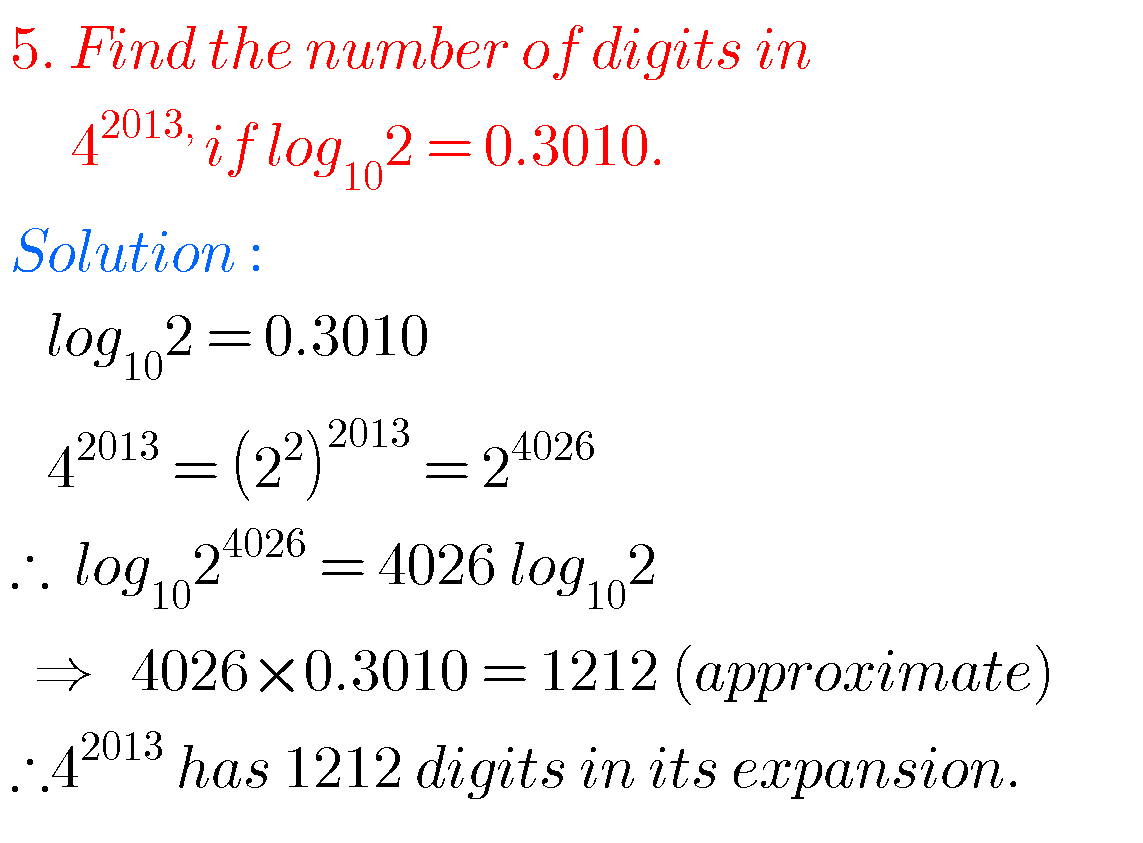Note :

Observe the solutions and try them in your own methods.

Inter maths solutions

Ncert maths solutions for some classes for some chapters